Browse by Topic

# Digital Vintage Sound: Modeling Analog Synthesizers with the Wolfram Language and System Modeler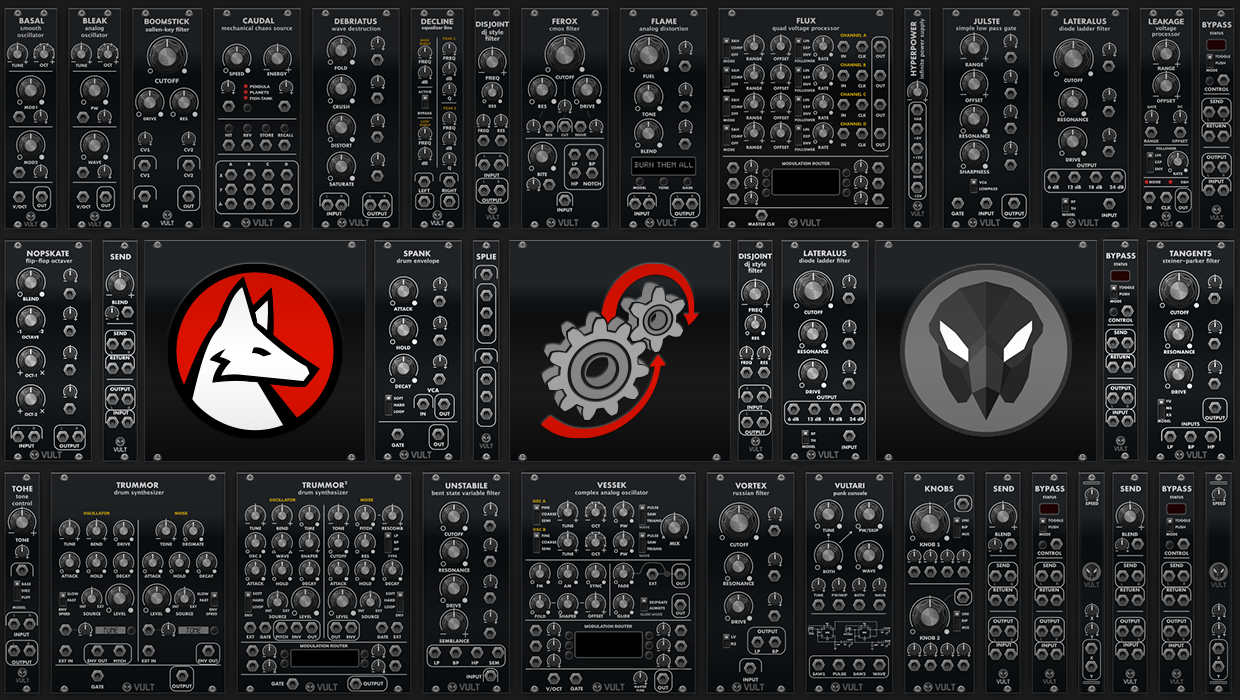Explore the contents of this article with a free Wolfram System Modeler trial. Have you ever thought about making your own musical instruments? What about making mathematical models of your instruments? Whether you’re someone looking for a cost-effective alternative, a minimalist with dreams of maximalist sounds or a Wolfram Language enthusiast curious about sound design, you can build a virtual version of a modular synthesizer using Wolfram System Modeler.

To give you a quick example of what’s possible with this technology, here’s a sample clip of techno-style music created entirely with simulation models:I use a modular synthesizer for designing sound and occasionally making songs, and I’ve found that System Modeler is ideal for creating a virtual synthesizer because it allows you to easily model and analyze the electric circuits used in vintage equipment.

## How Modular Synthesizers Work

My modular synthesizer is a mashup of different blocks: some of them were designed entirely by myself and others are commercial. How does a synthesizer like this work? Each module has a basic function. For example, it can be an oscillator, filter, envelope generator, amplifier, mixer, etc. By connecting these core components using wires, you can either imitate existing sounds or create new ones. You can see the similarities with System Modeler, where we can create complex systems by connecting components with virtual wires.

## Why Virtual?

Modular synthesizers are built in large and bulky cases. This makes them difficult to transport in a safe way. I have one small case that I carry when traveling, but it still uses a significant volume of my hand luggage.

Commercial modules can cost from as low as \$60 to over \$1,000 each, and a small synthesizer can have a dozen modules. That cost can add up quickly and become prohibitive for a hobbyist. If a person is handy with a soldering iron, it is possible to hand build many of the modules. In fact, there is a large community of enthusiasts like me who enjoy using and building these kinds of systems.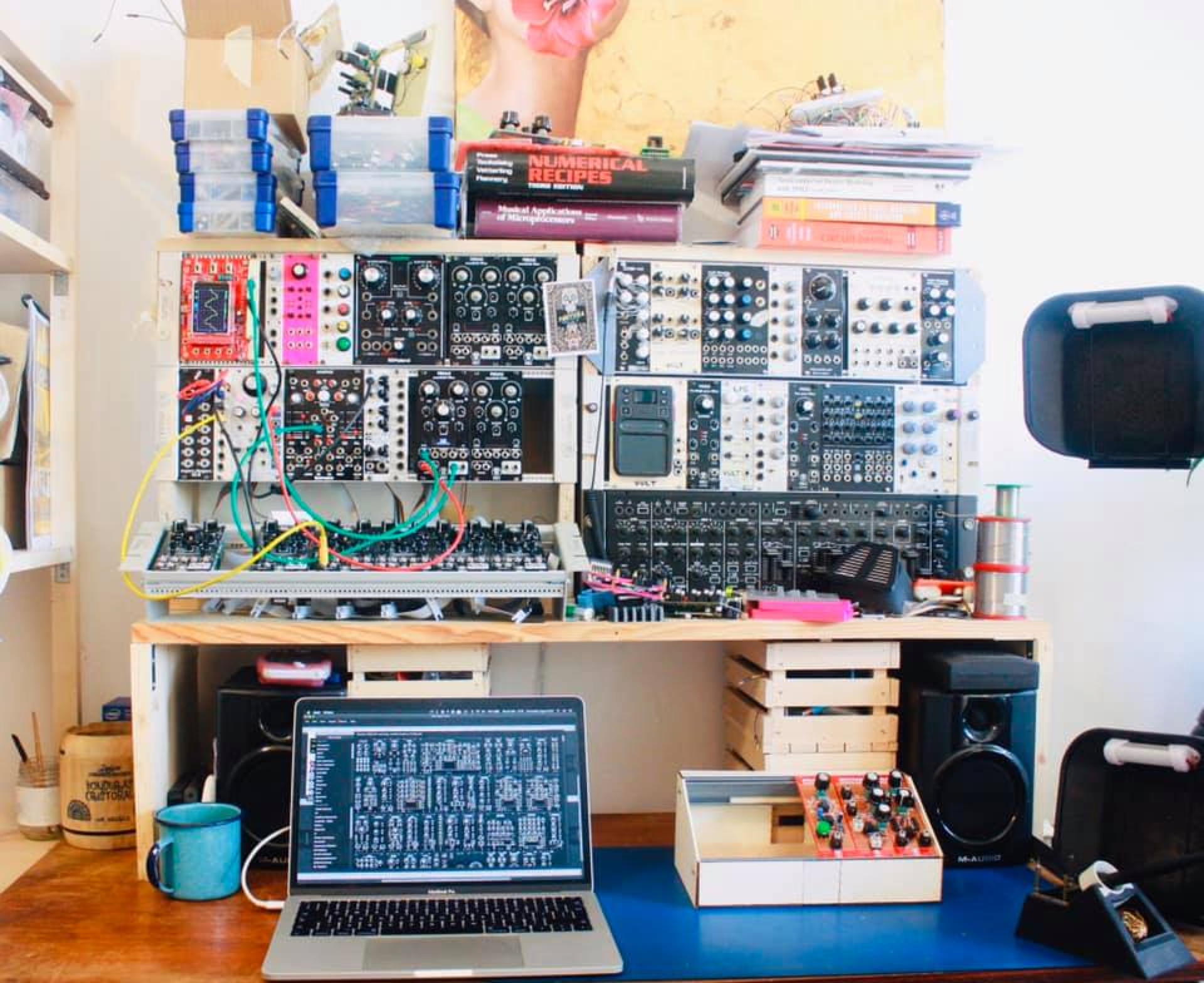My modular synthesizer, an instrument that I have been building component by component over the past few years.

There is, however, another alternative to experiencing a modular synthesizer at a low cost: virtual modular synthesizers. VCV Rack is one of the most popular open-source applications that aim to replicate the experience of using a modular synthesizer. It provides more than a thousand different modules.

Over the past two years, I have been releasing virtual modules for VCV Rack. One of the strong characteristics of my modules is that I try very hard to replicate in software the “analog vibes” of the sound. The analog modules are “imperfect,” in a good way. For example, a digital sine wave oscillator can produce a near-perfect signal. This perfect signal can be perceived as boring, lifeless or cold. On the other hand, an analog oscillator struggles to achieve that level of perfection but produces a sound that can be perceived as rich and warm.

I will explain some of the techniques I use to model and simulate analog circuits in order to create the digital counterparts that imitate the sound.

## The Basic Synthesizer Blocks

The sounds produced by a synthesizer can be completely new sounds or try to imitate existing ones. The most common technique used to create sounds in analog synthesizers is called subtractive synthesis, which involves starting with a rich sound and shaping (subtracting harmonics from) the sound in order to get the desired characteristics. You can think of it like having a piece of stone and then removing material in order to get the sculpture you want to create. In subtractive synthesis, the main modules are:

• Sound sources, for example any kind of oscillator that produces waves in the audible range
• Sound processors, for example filters and other effects
• Control sources; these can be low-frequency oscillators, envelope generators, etc.
• Utilities, such as amplifiers, attenuators, etc.

Let’s start modeling some basic modules: an oscillator and two filters. To help guide me in recreating an analog sound, I’ve selected these self-built modules from my collection to use as a reference: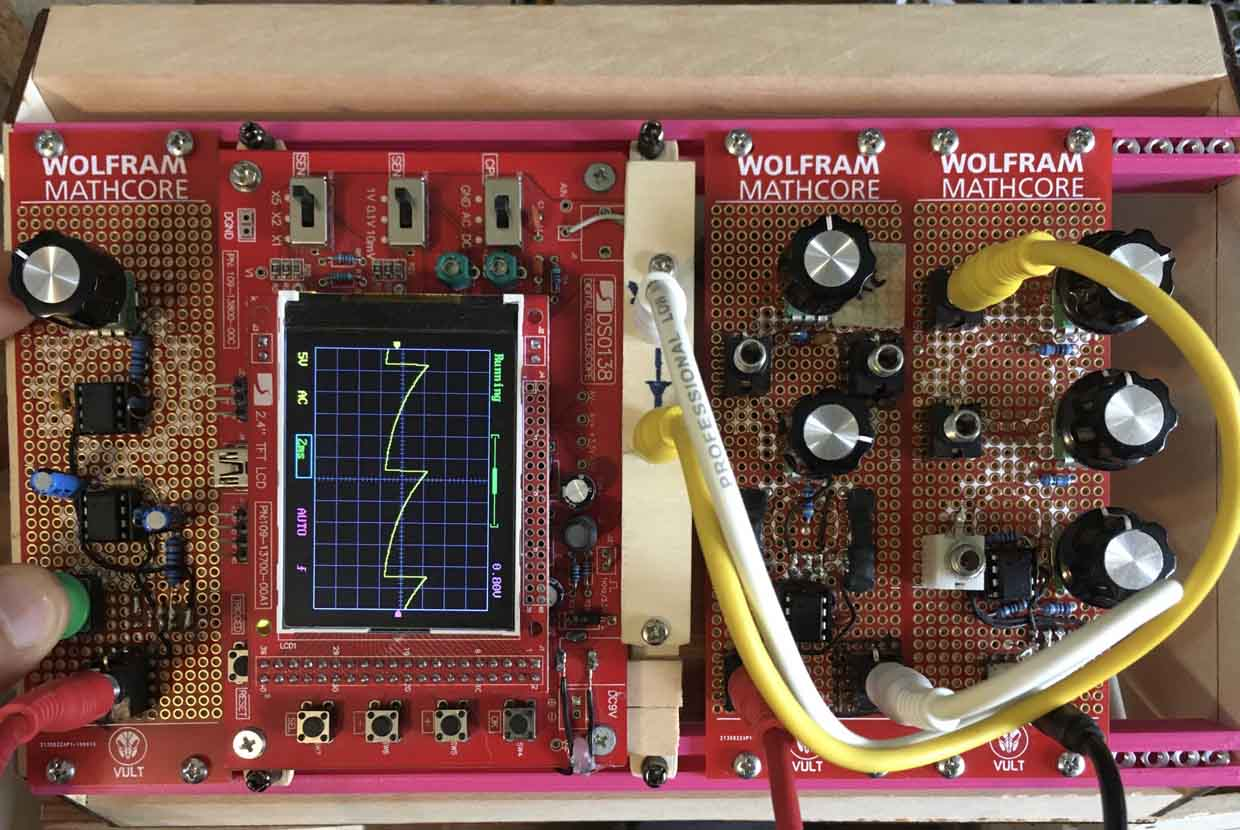A simple oscillator and two filters. The Wolfram MathCore team made these boards as souvenirs for the Wolfram Technology Conference in 2019, where some of the topics of this blog post were showcased.

## Modeling Basic Analog Components

In order to model these modules, we need to understand a bit how electric circuits are modeled. Let’s start with the most basic components: resistors and capacitors.

The commonly used electric components can be modeled using simple equations. Taking a look at the System Modeler code (by opening the Text View) for the built-in Resistor, we can see all the equations involved. One of the equations corresponds to Ohm’s law. The remaining equations are used to calculate the dissipated power and the effects of the temperature on the resistance. The equations for the terminals are described in the model OnePort. By modifying this code, we can create our custom electrical components for System Modeler: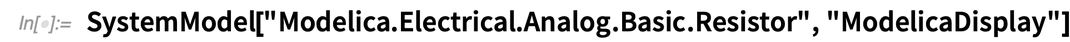✕ ```SystemModel["Modelica.Electrical.Analog.Basic.Resistor", \ "ModelicaDisplay"]```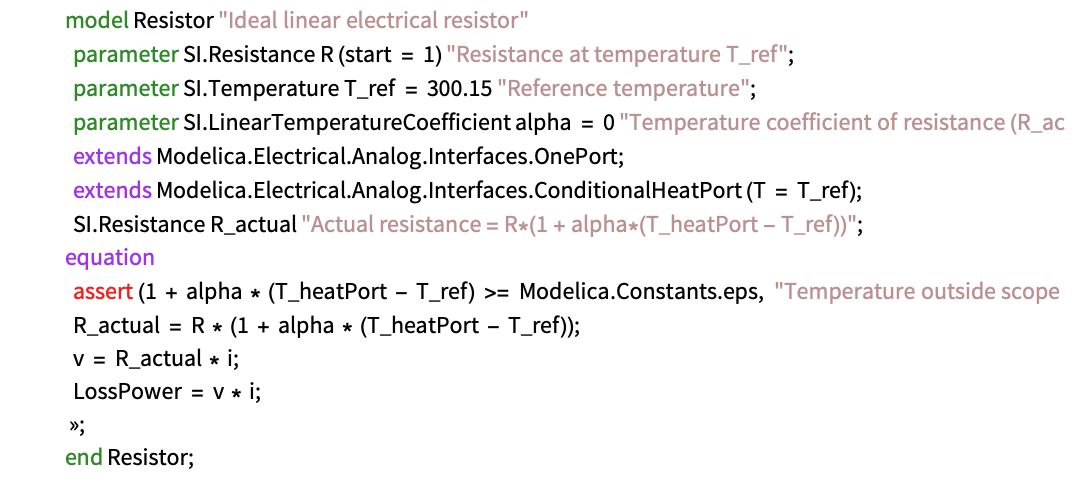Taking as a basis the resistor models shown previously, we can create a simplified version that models the effects that we are interested in and removes the ones that we will not use. (You can find all the models in the library AnalogModeling.) In our synthesizer models, we are going to assume that the components are at constant ambient temperature. We will remove as well any calculation of power dissipation since that would not have any effect on the sound. In the following listing, you can see the simplified resistor model that we created: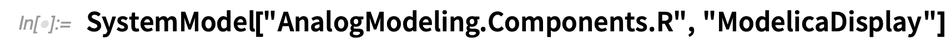✕ `SystemModel["AnalogModeling.Components.R", "ModelicaDisplay"]`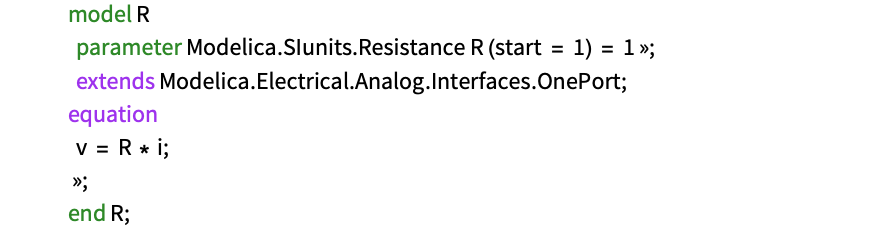The model of the capacitor is very similar. The main change is in the equation that defines the relation between the voltage and current. In the case of the capacitor, this is a differential equation. Next you can see the simplified capacitor model that we are going to use: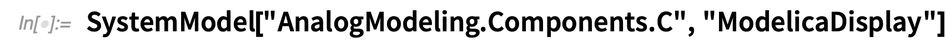✕ `SystemModel["AnalogModeling.Components.C", "ModelicaDisplay"]`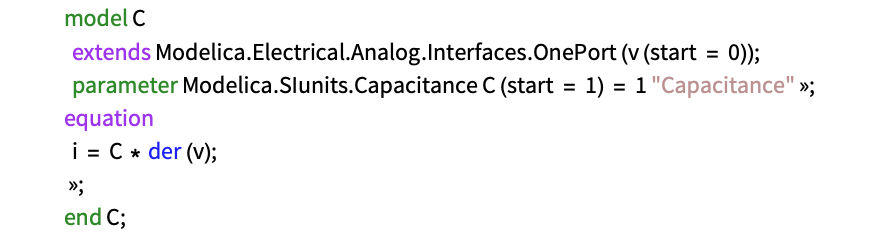Inductors are not common in the kind of audio circuits I model. For that reason, I’m not creating an inductor model right now, but it is very easy to derive from the capacitor model.

On the other hand, potentiometers are very common since they are the main elements that we use to control the parameters of synthesizers. To simulate potentiometers, I’ll first create a variable resistor. Once there is a variable resistor, I can combine two of them to create a three-pin potentiometer.

In order to control the position of the potentiometer, we will add an input signal u to the model. This signal goes from 0 to 1, which will correspond to the ranges of full counterclockwise and full clockwise motion, respectively: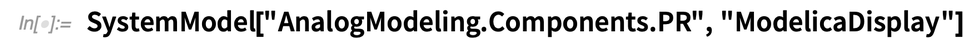✕ `SystemModel["AnalogModeling.Components.PR", "ModelicaDisplay"]`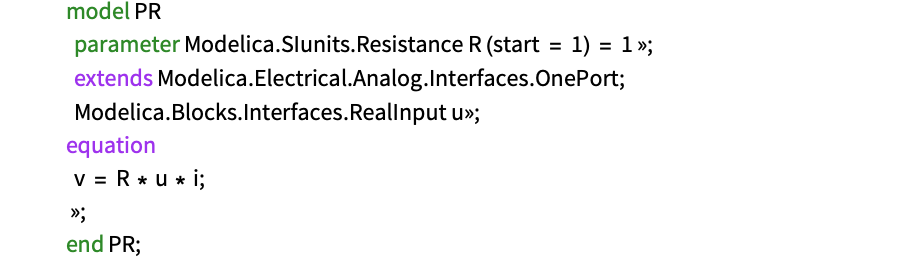Using two variable resistors, we can create a potentiometer by connecting it as shown in this diagram: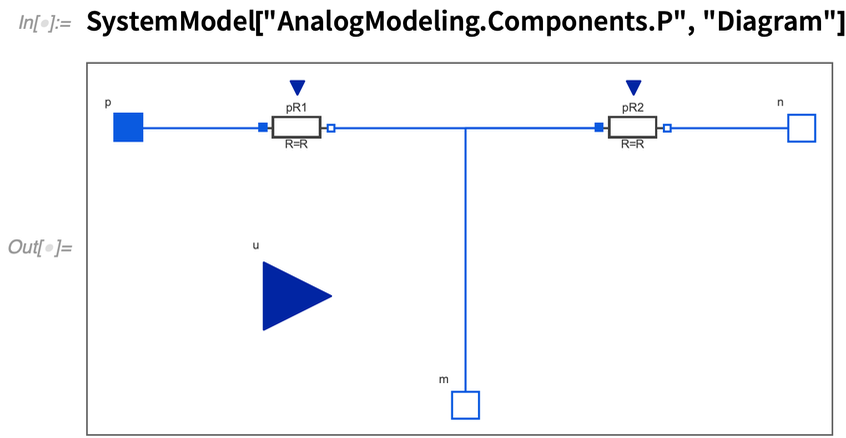✕ `SystemModel["AnalogModeling.Components.P", "Diagram"]`

The trick is to control both variable resistors using a single input. This is achieved by adding two equations to decrease the value of one resistor while the other increases: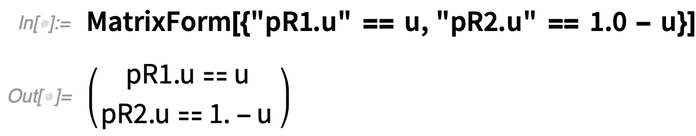✕ `MatrixForm[{"pR1.u" == u, "pR2.u" == 1.0 - u}]`

These equations affect the value of the resistors as shown in the following plot. The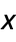axis has a range from 0 to 1: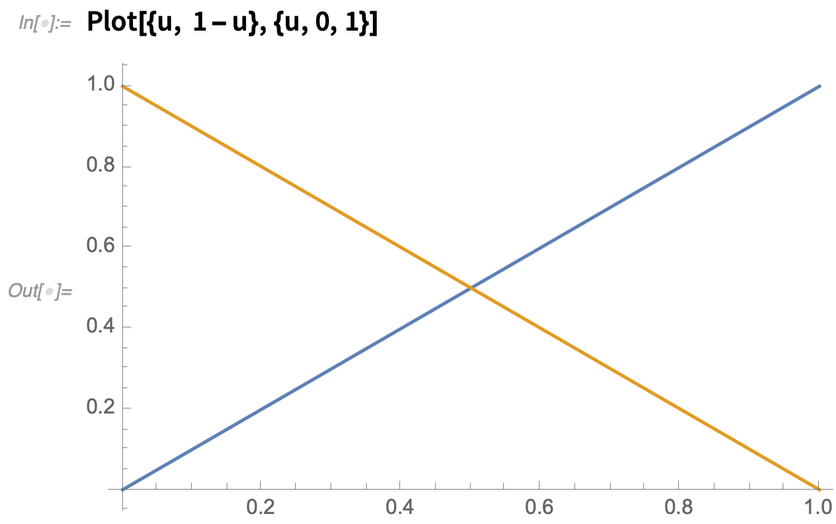✕ `Plot[{u, 1 - u}, {u, 0, 1}]`

Now that we have the basic components, let’s start modeling our first circuit.

## Modeling an RC Filter

Using the potentiometer and the capacitor created previously, we can easily wire an RC filter as follows: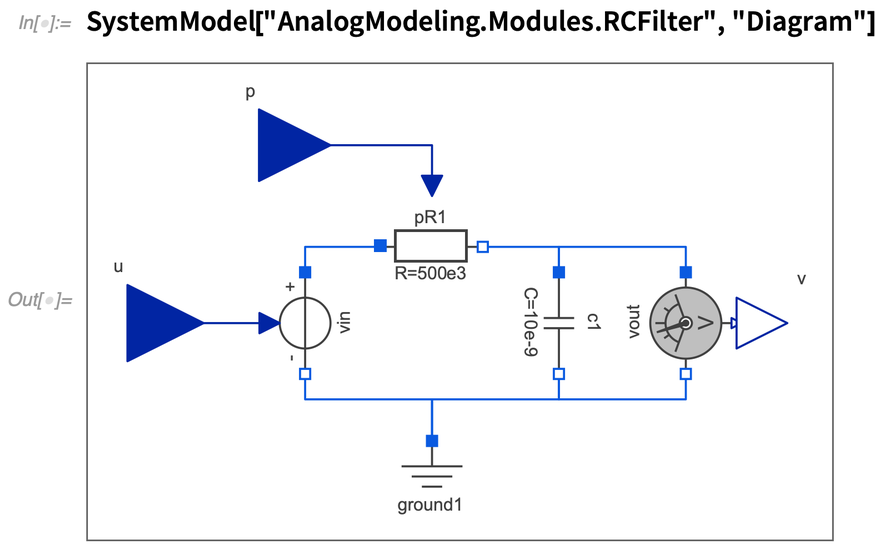✕ `SystemModel["AnalogModeling.Modules.RCFilter", "Diagram"]`

Notice the added inputs u and p to provide the input and control signals, respectively. The output v provides the voltage measured in the capacitor. We packed the RC filter model into a System Modeler component that we can reuse in different models.

In order to test the RC filter model, we used a few components from the System Modeler library that are used to provide stimulus signals: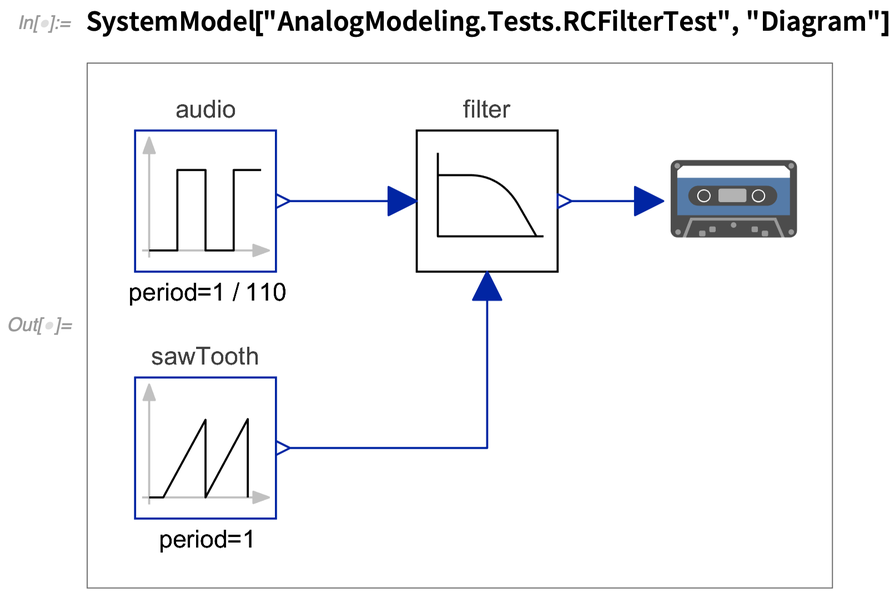✕ `SystemModel["AnalogModeling.Tests.RCFilterTest", "Diagram"]`

We used a pulse signal running at 110 Hz as audio input. A saw wave with a frequency of 1 Hz provides a signal that sets the position of the potentiometer. The output of the filter is then passed to a component that records the signal as a WAV file that can be played back to listen to the results.

We simulate our test model for four seconds: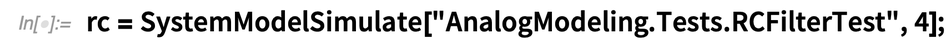✕ `rc = SystemModelSimulate["AnalogModeling.Tests.RCFilterTest", 4];`

The following plot shows the amplitudes of the input signal (in blue) and the filtered output (in orange) as we simulate a change in the position of the potentiometer: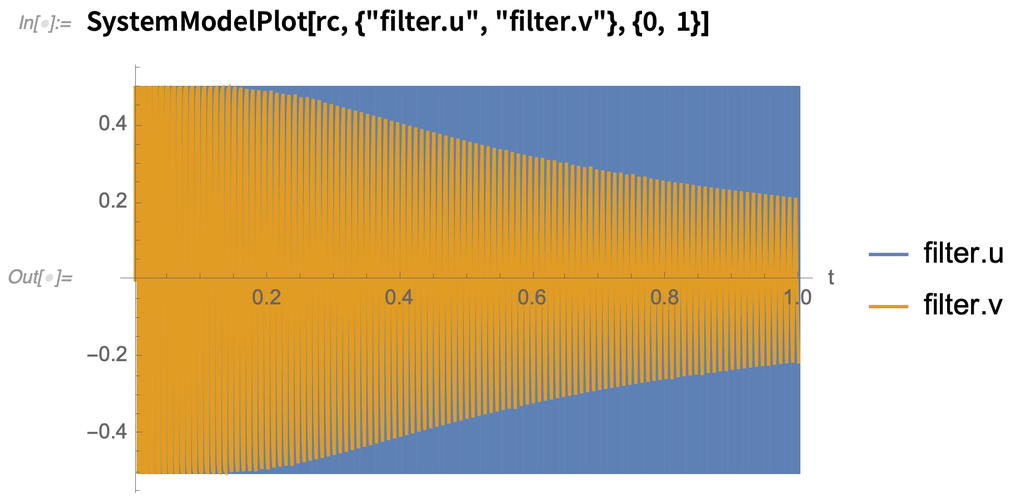✕ `SystemModelPlot[rc, {"filter.u", "filter.v"}, {0, 1}]`

We can see in the plot how the filter output is attenuated as the cutoff frequency of the filter is decreased. Initially, the filter is completely open, which means that the cutoff frequency is above the 22 kHz that humans are capable of hearing. As the potentiometer moves, the cutoff frequency is reduced and the filter attenuates more of the high-frequency harmonics of the signal.

An easier way to understand the effect is by listening to the sound produced by our test model. We can import the generated audio file and play it back. If you listen to the sound, you will hear how it changes as the RC filter removes the high harmonics. This sound resembles a string instrument that is plucked: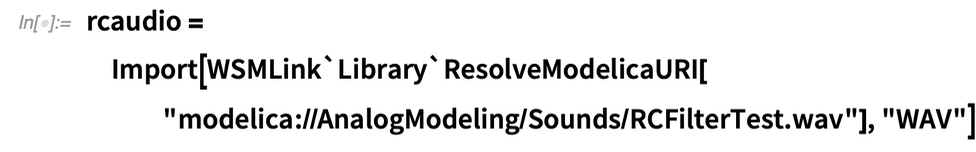✕ `rcaudio = Import[WSMLink`Library`ResolveModelicaURI["modelica://AnalogModeling/Sounds/RCFilterTest.wav"], "WAV"]`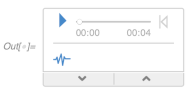Taking a look at the Spectrogram, the high-frequency harmonics (top of the graphic) are attenuated, while the low-frequency harmonics (bottom) are practically kept the same: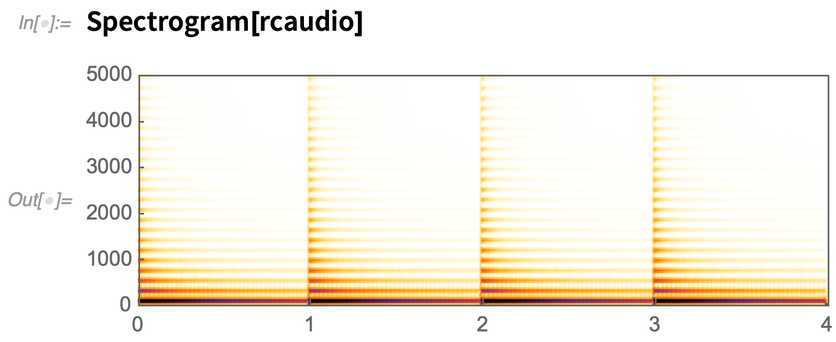✕ `Spectrogram[rcaudio]`

At this point, we have a model of an RC filter that allows us to run many analyses and provides us with very detailed information regarding the voltages and currents of every component. If we convert this model (as it is now) into an audio plugin, it would certainly work, but it would use more CPU than needed. This is because our model has many equations that calculate all the details of the circuit. However, when we are trying to make music, we are not very concerned about the internals of the circuit; the filter is more like a black box. In order to make the model more efficient, we can use the symbolic capabilities of the Wolfram Language to minimize our set of equations. Our target is to obtain the ODE representation of the circuit.

Our simple RC filter consists of 27 equations. We can see them all using the SystemModel command: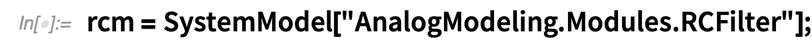✕ `rcm = SystemModel["AnalogModeling.Modules.RCFilter"];`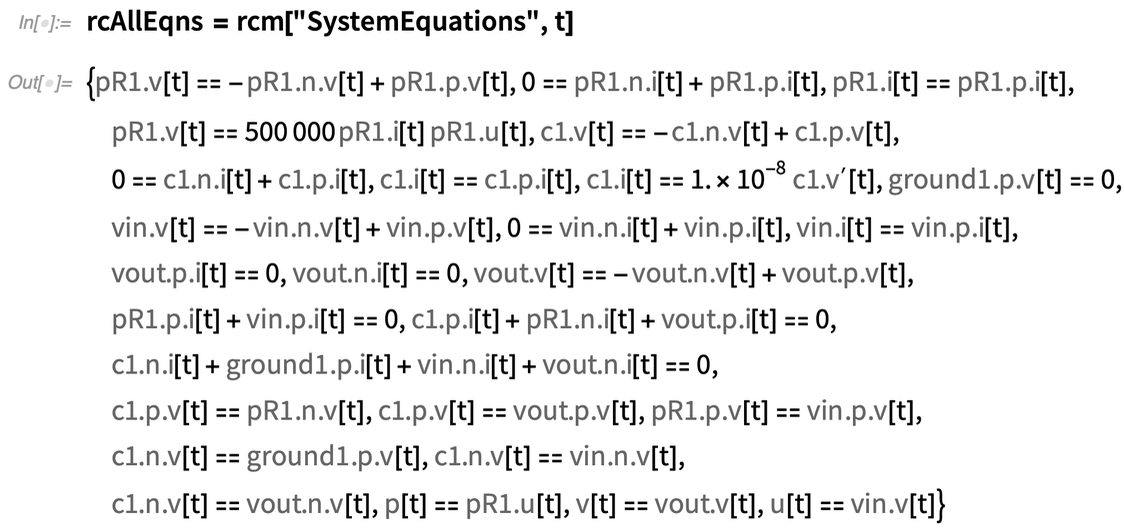✕ `rcAllEqns = rcm["SystemEquations", t]`

The SytemModel command can help us in reducing the number of equations if we specify the level of elimination as follows: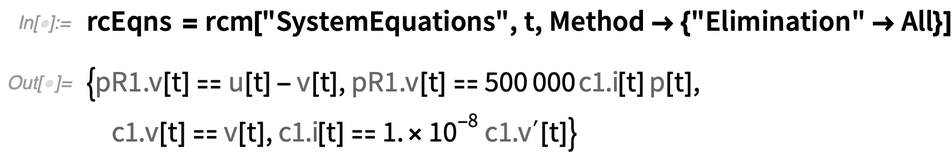✕ `rcEqns = rcm["SystemEquations", t, Method -> {"Elimination" -> All}]`

We can see that the number of equations was reduced to four. This is achieved by eliminating all the trivial equations like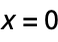and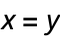. In this case, we are intentionally losing information about our model since we are not able to calculate all voltages and currents of the circuit anymore. The resulting set of equations can be reduced further by using the command Eliminate. While eliminating the last variables, we can also replace some of the variable names with short symbols that will improve the readability of the equations. This simplification results in a single differential equation where the name dvc represents the derivative of vc (voltage of the capacitor), and vin is the input voltage: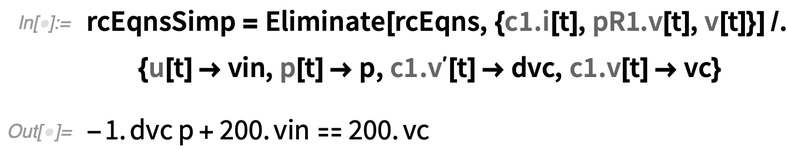✕ ```rcEqnsSimp = Eliminate[ rcEqns, {QuantityVariable["c1.i","ElectricCurrent"][t], QuantityVariable["pR1.v","ElectricPotential"][t], QuantityVariable["v",""][t]}] /. {QuantityVariable["u",""][t] -> vin, QuantityVariable["p",""][t] -> p, Derivative[QuantityVariable["c1.v","ElectricPotential"]][t] -> dvc, QuantityVariable["c1.v","ElectricPotential"][t] -> vc}```

We can take the resulting equation and use the command Solve to put it in a traditional ODE form: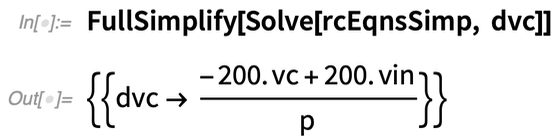✕ `FullSimplify[Solve[rcEqnsSimp, dvc]]`

From this differential equation, the transfer function of the filter is easily obtained: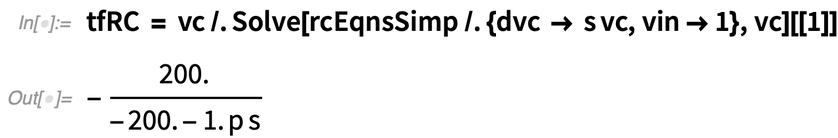✕ `tfRC = vc /. Solve[rcEqnsSimp /. {dvc -> s vc, vin -> 1}, vc][]`

In order to analytically confirm the behavior of the filter, we can create the Bode plot of the transfer function for three different positions of the potentiometer. In this plot, we can see what would be the attenuation of the input audio signal depending on its frequency content: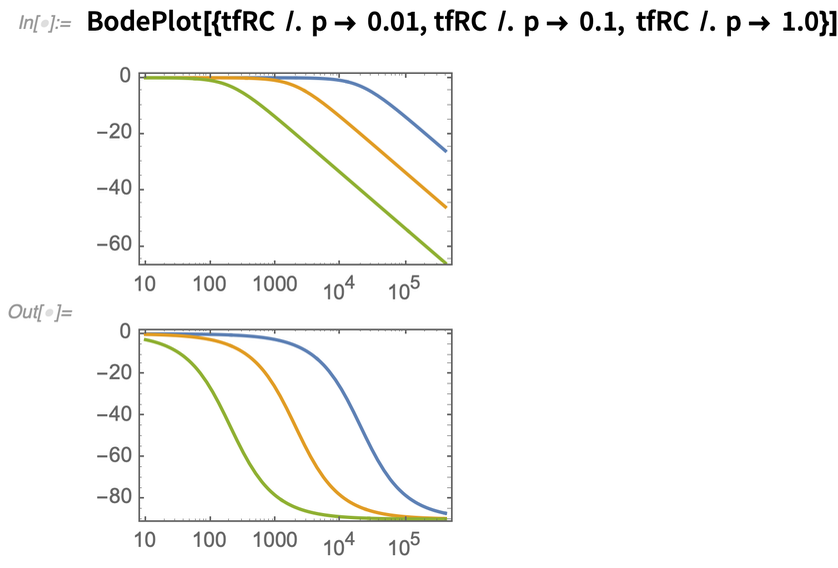✕ `BodePlot[{tfRC /. p -> 0.01, tfRC /. p -> 0.1, tfRC /. p -> 1.0}]`

This equation is a simple differential equation that can be easily implemented with any programming language. Later, I’ll discuss how to implement it and analyze difficulties that can arise.

For now, let’s model a slightly more complex filter.

## Modeling a Sallen–Key Filter

The Sallen–Key topology allows building different kinds of filters, for example lowpass, highpass, bandpass, etc. I will focus on the lowpass configuration for now. The previously modeled simple RC filter has one pole, while the Sallen–Key filter has two poles and therefore is “twice” as effective at removing frequencies: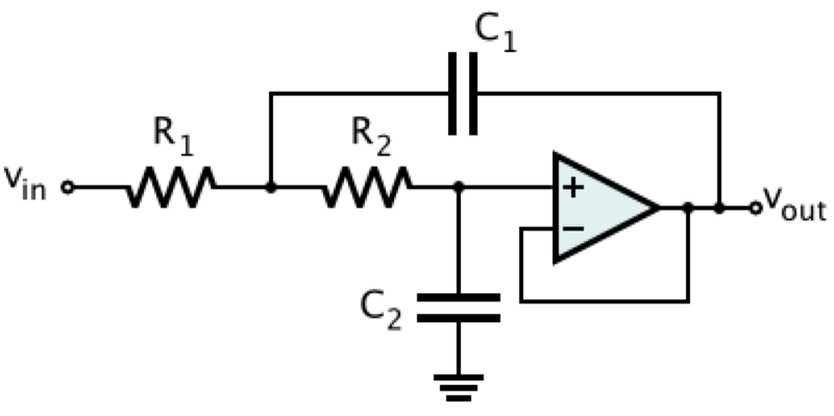The previous diagram shows that that an operational amplifier (OPAMP) is needed to build this model. In this case, the OPAMP is connected in follower configuration, which makes it very easy to create a basic model. In follower mode, the output voltage of the OPAMP is exactly the input voltage, but the input current is practically zero. This configuration is known as the buffer.

A simple model for the buffer can be made in System Modeler using components from the Modelica library. Notice that this model is very simple and does not consider nonlinear behaviors of a real OPAMP—for example, output voltage saturation. To get a more analog sound, I would have to model saturation and other effects that we will not cover in this blog post.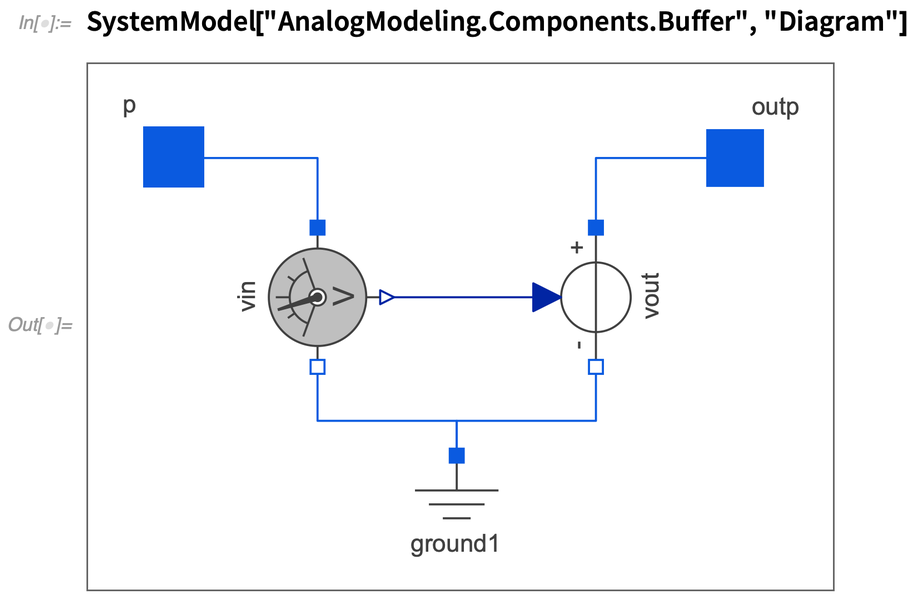✕ `SystemModel["AnalogModeling.Components.Buffer", "Diagram"]`

Once we have a model of the buffer, a simulation model of the Sallen–Key filter can be built by connecting the components. In order to change the frequency, we need a dual potentiometer. Dual potentiometers, as the name implies, are two individual potentiometers whose positions are controlled by a single shaft. In the Sallen–Key filter model, we will use two potentiometer components and control them using the same signal.

You can see the schematic of our new filter component in the following figure: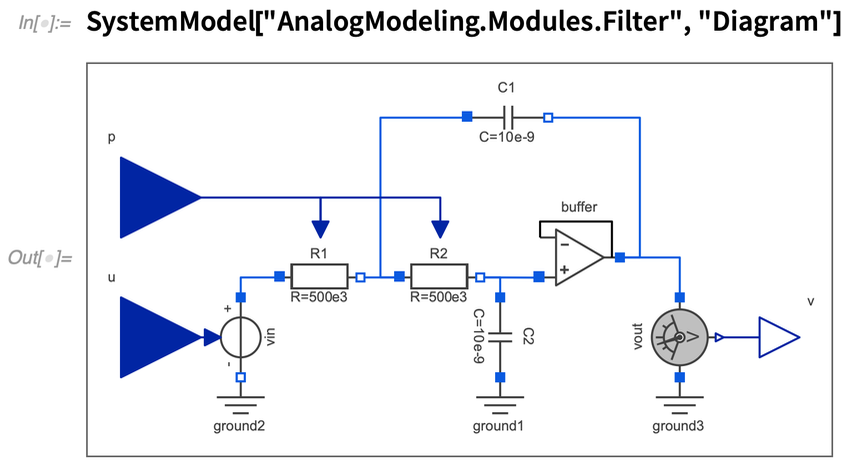✕ `SystemModel["AnalogModeling.Modules.Filter", "Diagram"]`

Now let’s simulate a filter using a model similar to the one we used to test the RC filter (a pulse wave as audio and a saw wave to control the potentiometer). We simulate the test model for four seconds and plot the results: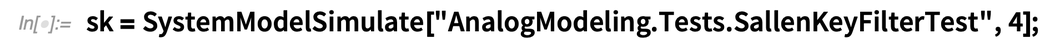✕ ```sk = SystemModelSimulate["AnalogModeling.Tests.SallenKeyFilterTest", 4];```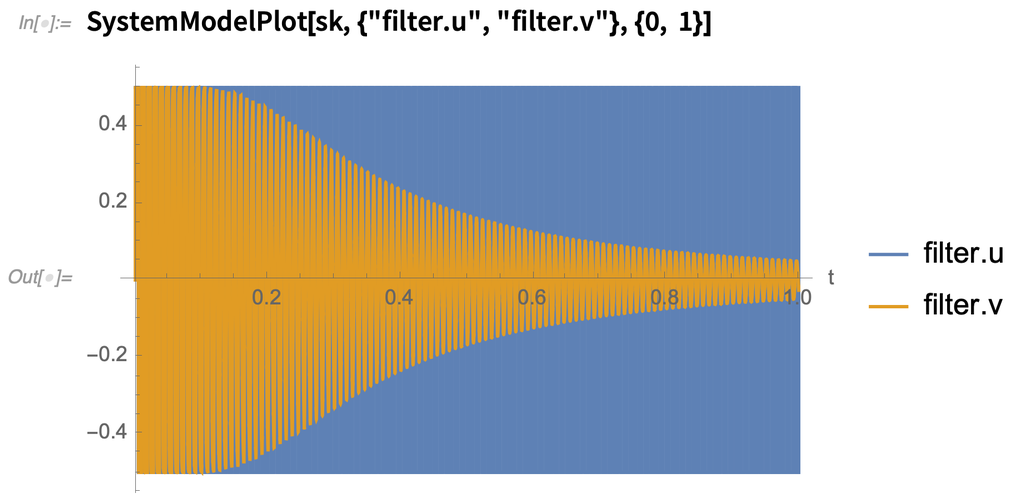✕ `SystemModelPlot[sk, {"filter.u", "filter.v"}, {0, 1}]`

Again, the orange signal is the filtered output and the blue is the audio input. We can listen to the sound of this filter in the following audio clip: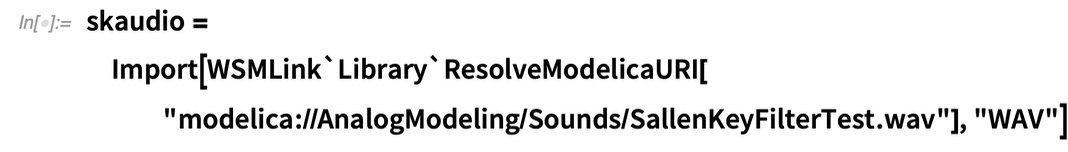✕ ```skaudio = Import[WSMLink`Library`ResolveModelicaURI[ "modelica://AnalogModeling/Sounds/SallenKeyFilterTest.wav"], "WAV"]```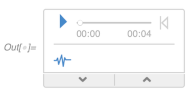You may notice that the result still sounds like plucking a string instrument, but the sound is a bit more mellow. This is because this filter removes more of the high-frequency harmonics. This effect can be seen in the spectrogram plots. Next, you can see the output of the Sallen–Key and RC filters: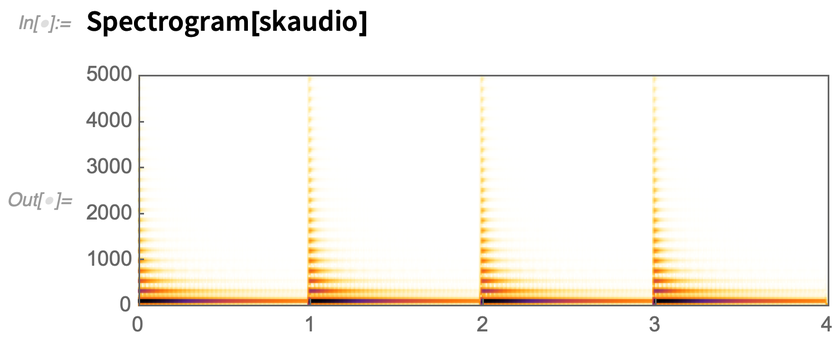✕ `Spectrogram[skaudio]`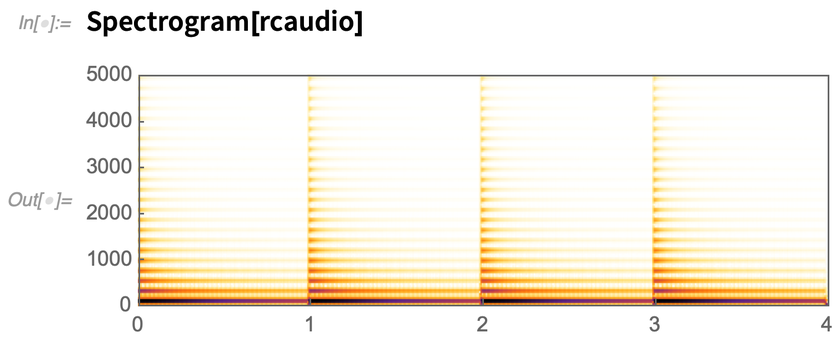✕ `Spectrogram[rcaudio]`

Now that we have a working Sallen–Key filter, let’s follow the same simplification approach in order to get the differential equations and the transfer function. Eliminate all the unneeded variables and replace the names with shorter variables: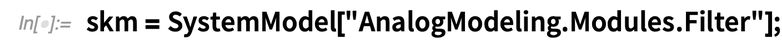✕ `skm = SystemModel["AnalogModeling.Modules.Filter"];`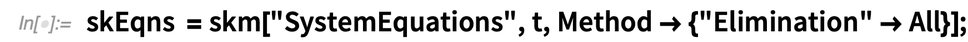✕ `skEqns = skm["SystemEquations", t, Method -> {"Elimination" -> All}];`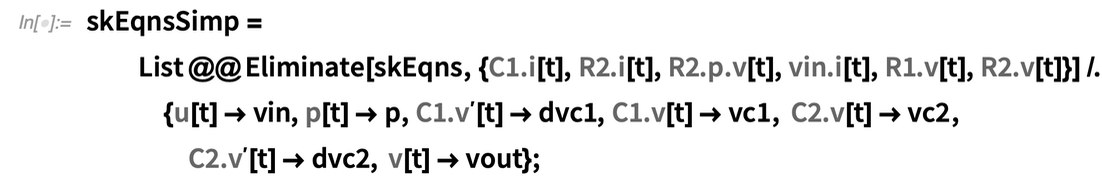✕ ```skEqnsSimp = List @@ Eliminate[ skEqns, {QuantityVariable["C1.i","ElectricCurrent"][t], QuantityVariable["R2.i","ElectricCurrent"][t], QuantityVariable["R2.p.v","ElectricPotential"][t], QuantityVariable["vin.i","ElectricCurrent"][t], QuantityVariable["R1.v","ElectricPotential"][t], QuantityVariable["R2.v","ElectricPotential"][ t]}] /. {QuantityVariable["u",""][t] -> vin, QuantityVariable["p",""][t] -> p, Derivative[QuantityVariable["C1.v","ElectricPotential"]][t] -> dvc1, QuantityVariable["C1.v","ElectricPotential"][t] -> vc1, QuantityVariable["C2.v","ElectricPotential"][t] -> vc2, Derivative[QuantityVariable["C2.v","ElectricPotential"]][t] -> dvc2, QuantityVariable["v",""][t] -> vout};```

The system has two differential equations, one for each capacitor. The output voltage corresponds to the voltage of the capacitor C2: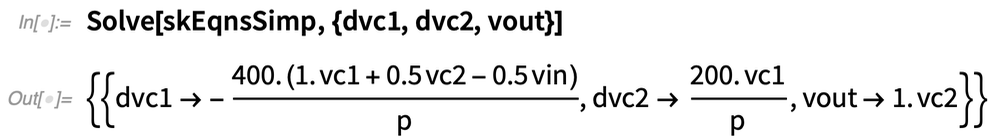✕ `Solve[skEqnsSimp, {dvc1, dvc2, vout}]`

From those differential equations, obtain the transfer function in terms of the potentiometer position: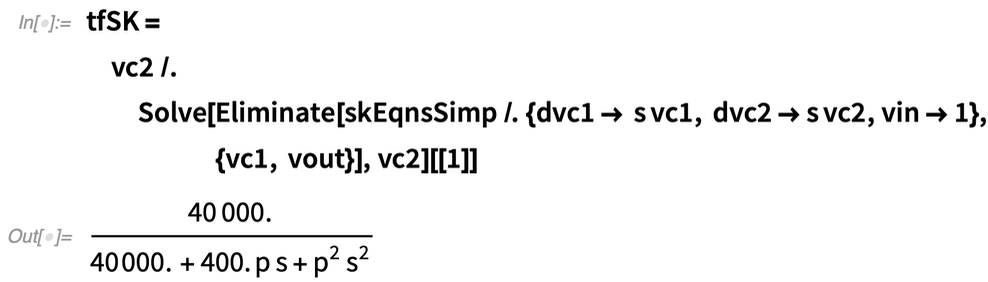✕ ```tfSK = vc2 /. Solve[Eliminate[ skEqnsSimp /. {dvc1 -> s vc1, dvc2 -> s vc2, vin -> 1}, {vc1, vout}], vc2][]```

The following plot shows the frequency response for three different positions of the potentiometer: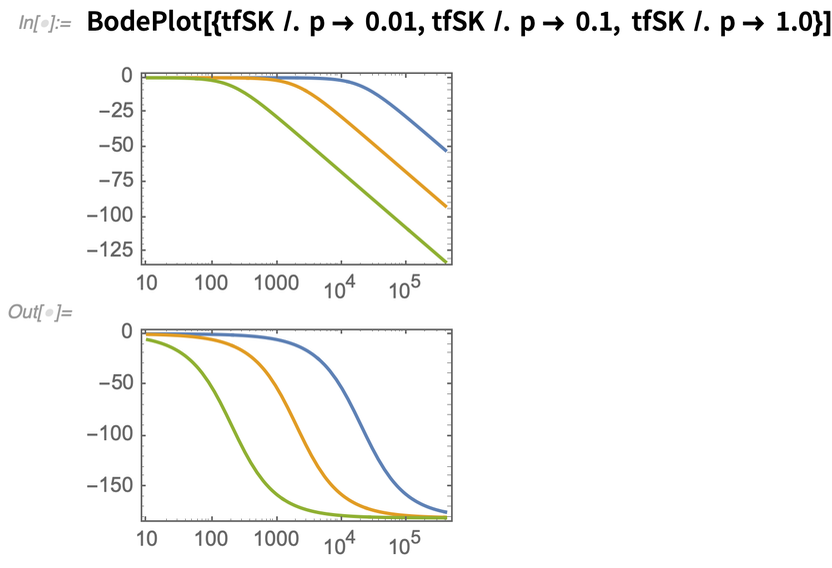✕ `BodePlot[{tfSK /. p -> 0.01, tfSK /. p -> 0.1, tfSK /. p -> 1.0}]`

Now let’s make a side-by-side comparison of the two filters. Next you can see the frequency response of both filters: the Sallen–Key in orange and the RC in blue. The RC filter is a single pole that provides an attenuation of 20 dB per decade (6 dB per octave), while the Sallen–Key filter provides 40 dB of attenuation (12 dB per octave):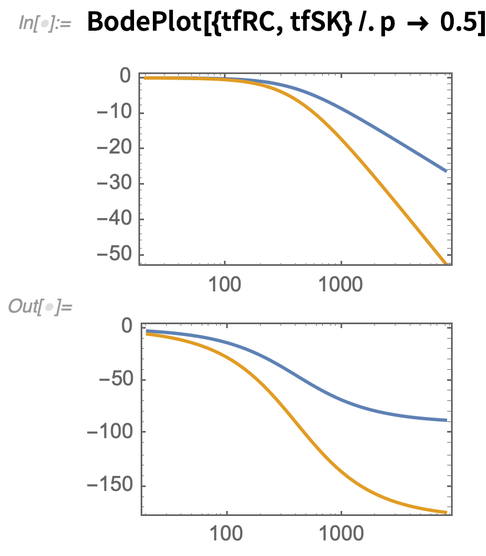✕ `BodePlot[{tfRC, tfSK} /. p -> 0.5]`

## Modeling a Simple Oscillator

The DIY oscillator that we are using as reference is built using the 555 integrated circuit. Usually, oscillators based on the 555 are pulse generators. In our case, we have extended the circuit to produce a saw wave oscillator. To simulate this oscillator, we need to create a model of the 555 IC first. Luckily, the 555 is very well documented and we can easily find a block diagram describing all the internals of the IC: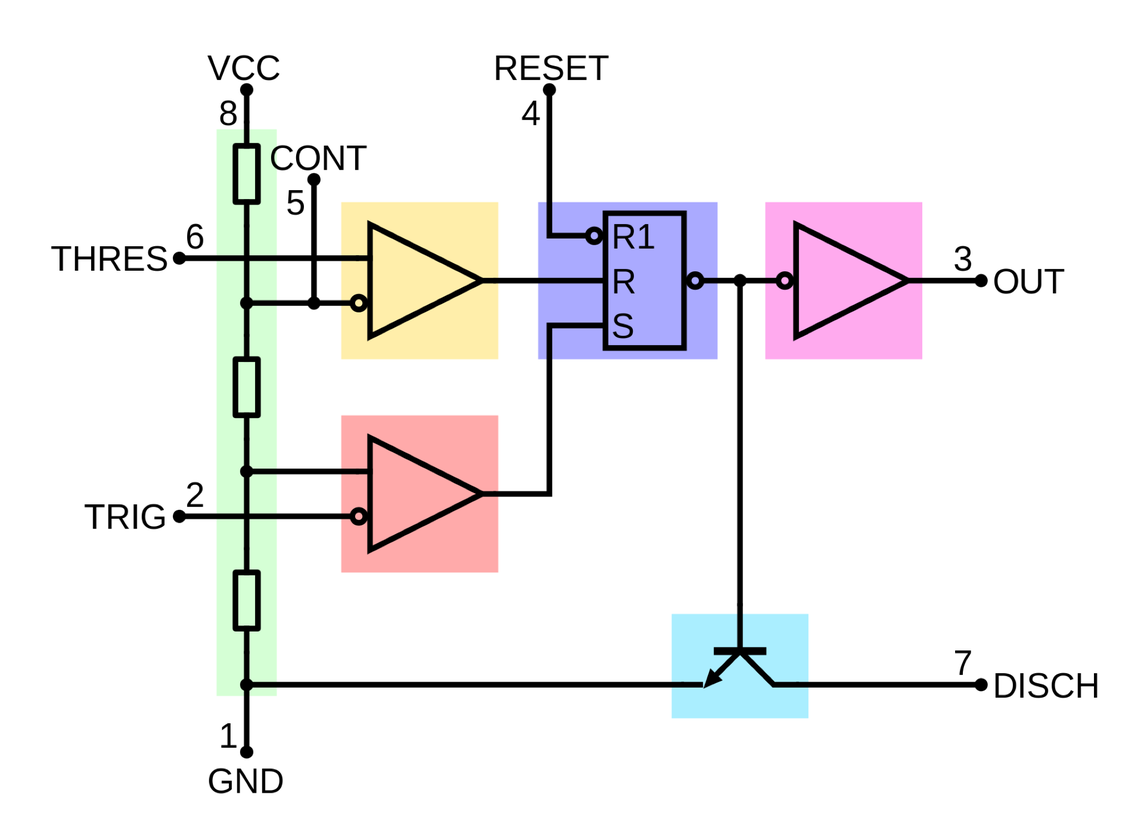The 555 consists of a voltage divider, two comparators, one flip-flip, one transistor and a buffer. I could create this model using Modelica components. However, in this case, I decided to experiment by creating the model directly in Modelica code. This is one big feature that System Modeler provides for advanced users or library developers who want to have full control of the equations involving their models.

Here we can see the code I created. It is not important for the reader to understand it. Just know that this code is an equivalent to the block diagram that was presented earlier.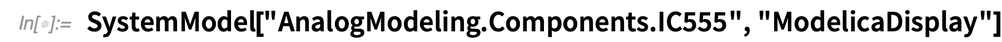✕ `SystemModel["AnalogModeling.Components.IC555", "ModelicaDisplay"]`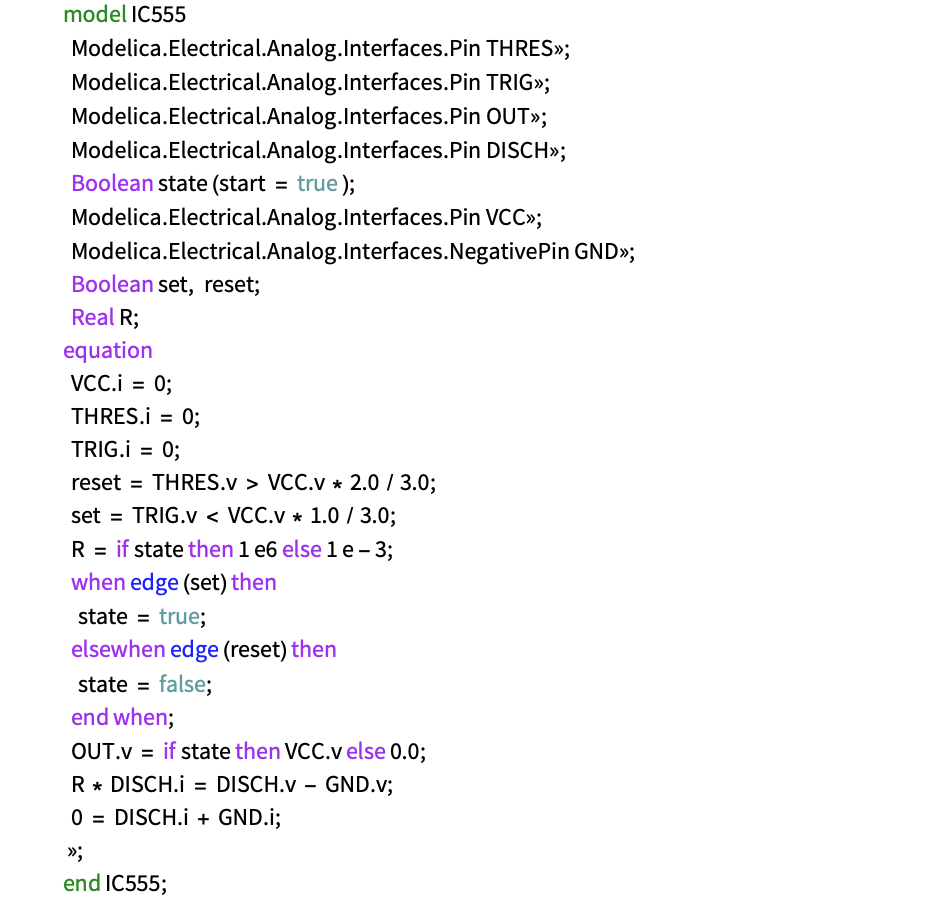The oscillator is made by connecting the 555 in the oscillator configuration commonly called “astable.” This will allow us to control the pitch using a single potentiometer. To generate the saw wave, we used a buffer to measure the voltage of the charge/discharge capacitor C1 and then we added C2 as a decoupling capacitor to remove the DC offset. You can see the diagram of the oscillator component in the following figure: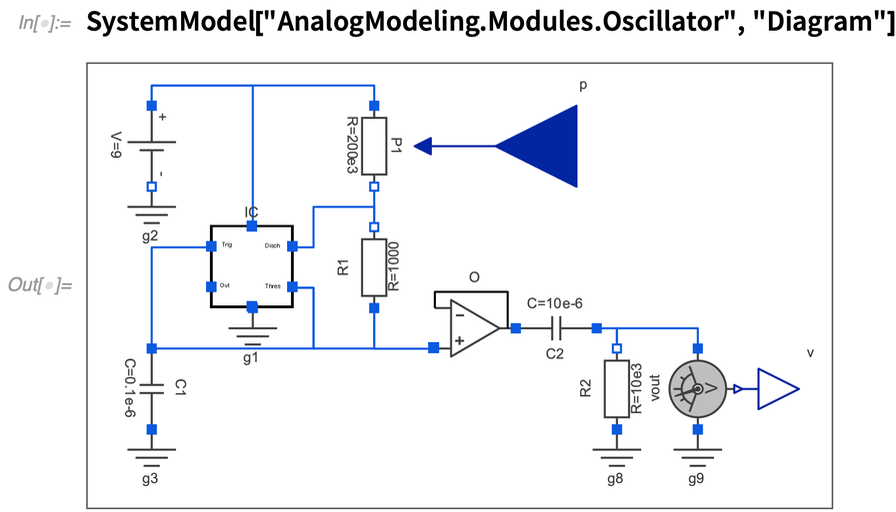✕ `SystemModel["AnalogModeling.Modules.Oscillator", "Diagram"]`

To test this model, we used a ramp that simulates the turning of the potentiometer, resulting in changing the pitch of the oscillator. Similar to the previous test performed on the filters, we recorded the audio produced by the simulation: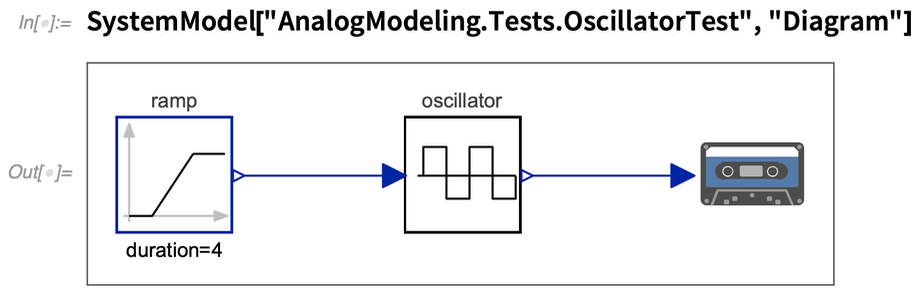✕ `SystemModel["AnalogModeling.Tests.OscillatorTest", "Diagram"]`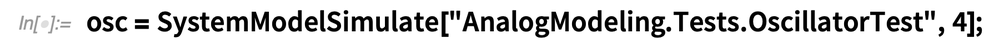✕ `osc = SystemModelSimulate["AnalogModeling.Tests.OscillatorTest", 4];`

If we plot a small segment of the simulation results, we can see the produced saw wave: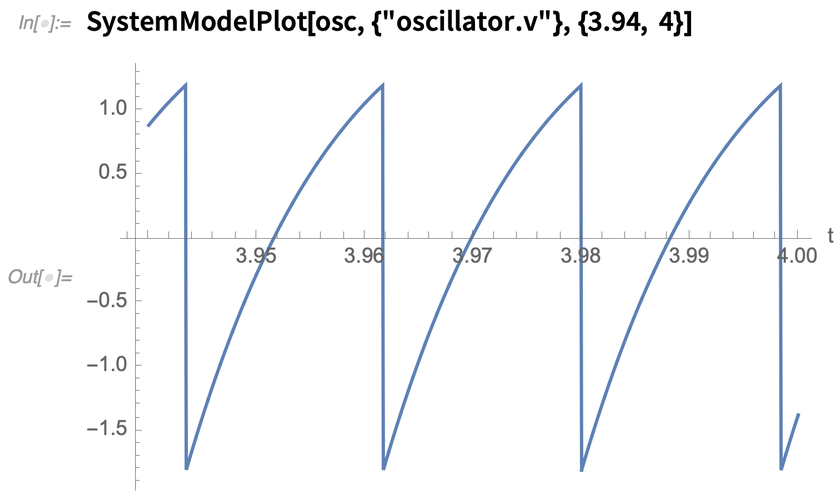✕ `SystemModelPlot[osc, {"oscillator.v"}, {3.94, 4}]`

The first thing you may notice is that the wave is not a perfect saw. This is in part because the charging of the capacitor is not perfectly linear. These kinds of small details are what make analog oscillators sound the way they do. However, not all imperfections of analog oscillators are desired. For example, the analog oscillators can detune with the temperature. To compensate for that, more sophisticated circuits can include temperature compensation.

When importing the produced audio file, we can hear how the oscillator sounds: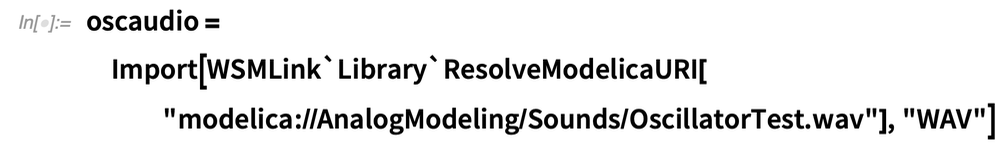✕ ```oscaudio = Import[WSMLink`Library`ResolveModelicaURI[ "modelica://AnalogModeling/Sounds/OscillatorTest.wav"], "WAV"]```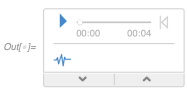We can hear that the sound starts with a high pitch and gradually changes into a lower frequency. We can see this effect in the spectrogram. One more thing to notice is how the saw wave is comprised of many harmonics that make it a rich signal: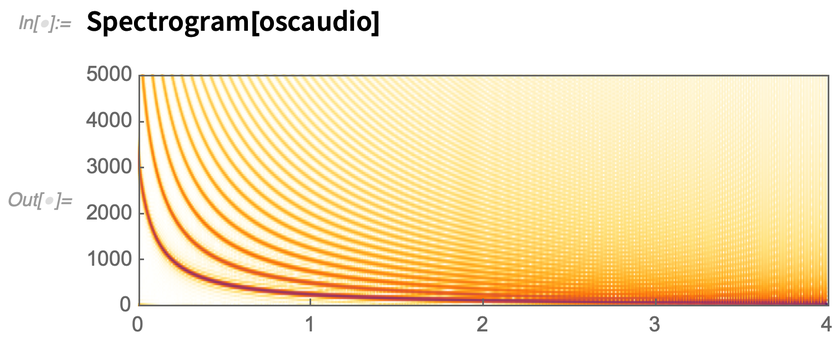✕ `Spectrogram[oscaudio]`

As mentioned earlier, subtractive synthesis consists of shaping the harmonics by using modules like filters. Now let’s find out how the combination of our filter and oscillator sounds.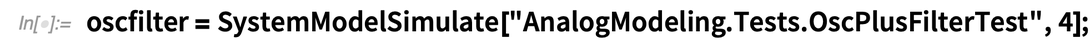✕ ```oscfilter = SystemModelSimulate["AnalogModeling.Tests.OscPlusFilterTest", 4];```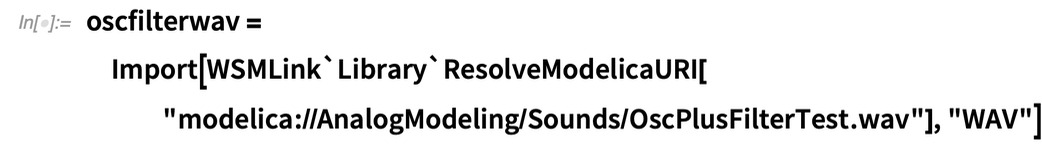✕ ```oscfilterwav = Import[WSMLink`Library`ResolveModelicaURI[ "modelica://AnalogModeling/Sounds/OscPlusFilterTest.wav"], "WAV"]```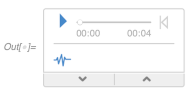The resulting sound resembles a tuba. By plotting the spectrogram, we can see how the filter opens and closes, removing many of the harmonics.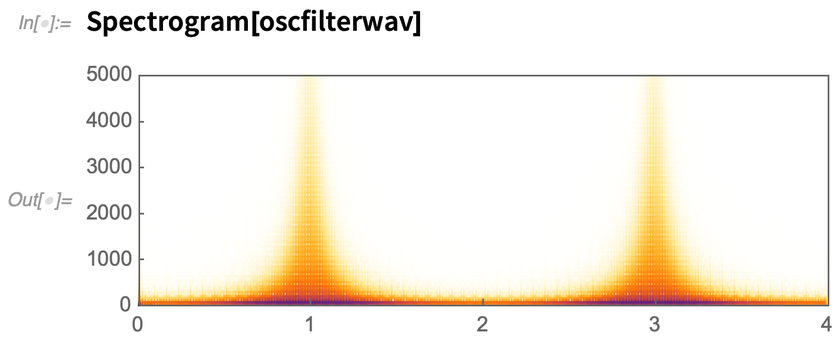✕ `Spectrogram[oscfilterwav]`

## Simulating Other Synthesizer Modules

So far, we’ve discussed two basic modules, an oscillator and a filter. In order to create more complex sounds, models must be created for some of the common utility functions, like envelope generators and amplifiers. The following components are not modeled after existing circuits. They are behavioral models that imitate the actual behavior of the real module.

The first one is the VCA, which stands for voltage-controlled amplifier. Implementing a VCA in System Modeler is straightforward because a VCA is a multiplication of two signals. One of the signals is used as a control and the other as a source.

Next is a diagram and the icon of the VCA: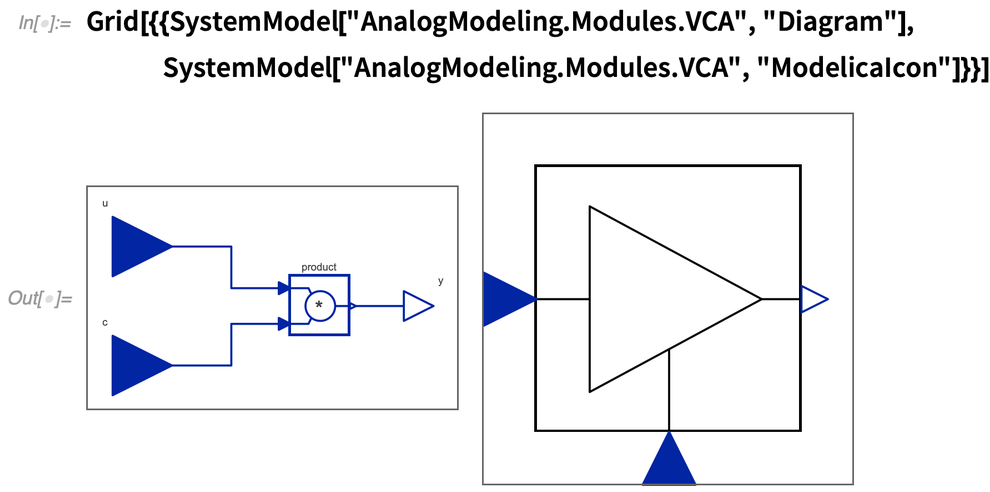✕ ```Grid[{{SystemModel["AnalogModeling.Modules.VCA", "Diagram"], SystemModel["AnalogModeling.Modules.VCA", "ModelicaIcon"]}}]```

We can test this model by using two sine wave components from the Modelica library: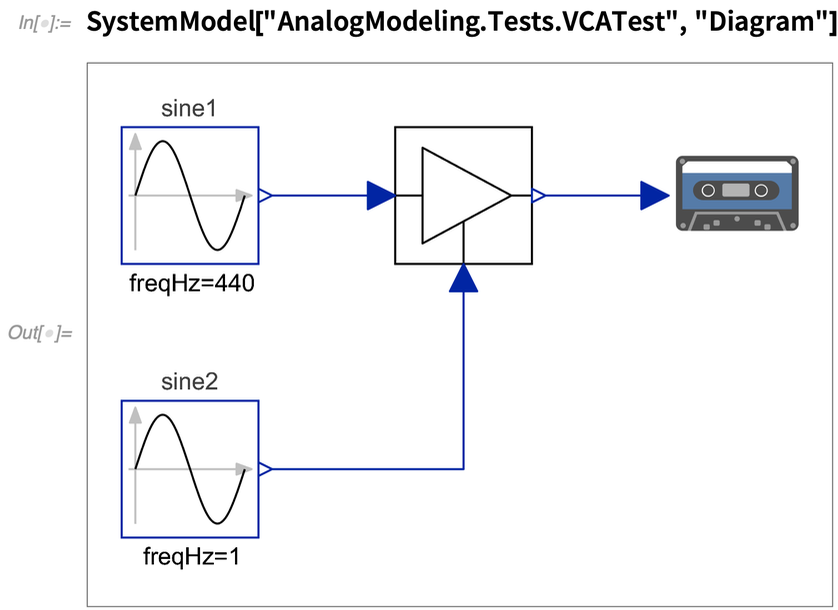✕ `SystemModel["AnalogModeling.Tests.VCATest", "Diagram"]`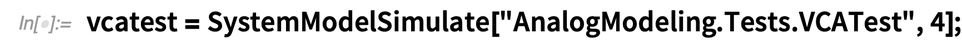✕ `vcatest = SystemModelSimulate["AnalogModeling.Tests.VCATest", 4];`

In the simulation result, we can see the change of amplitude of the signal: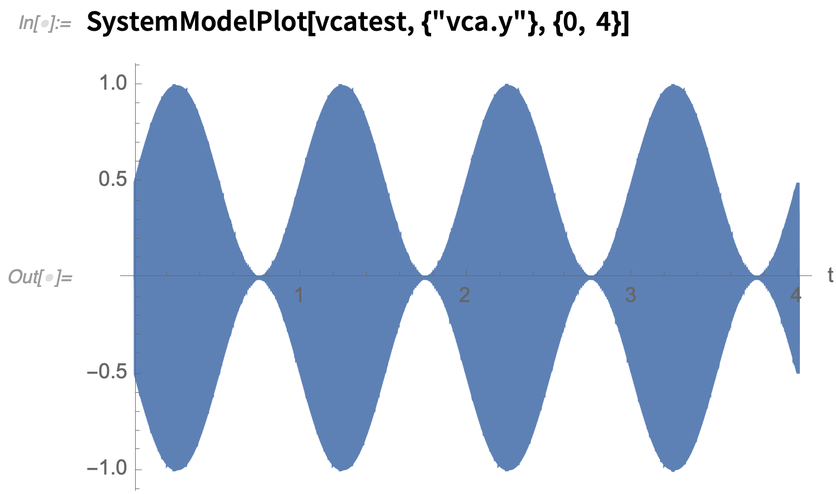✕ `SystemModelPlot[vcatest, {"vca.y"}, {0, 4}]`

If you listen to the audio, you will notice how the perceived volume changes: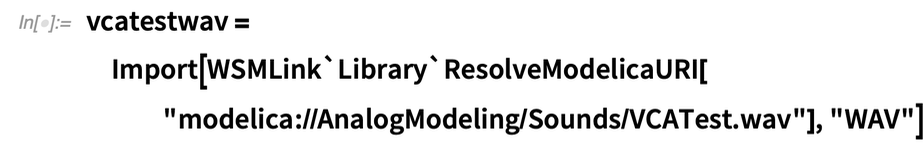✕ ```vcatestwav = Import[WSMLink`Library`ResolveModelicaURI[ "modelica://AnalogModeling/Sounds/VCATest.wav"], "WAV"]```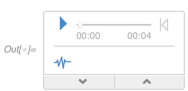The next model that we need is an envelope generator. Envelopes are commonly used to shape the amplitude of the sound. For example, when hitting a drum, the sound has initially a sudden rise of amplitude, then it gradually decays. To model that behavior, we can use a simple RC circuit that is very similar to the filter we presented before: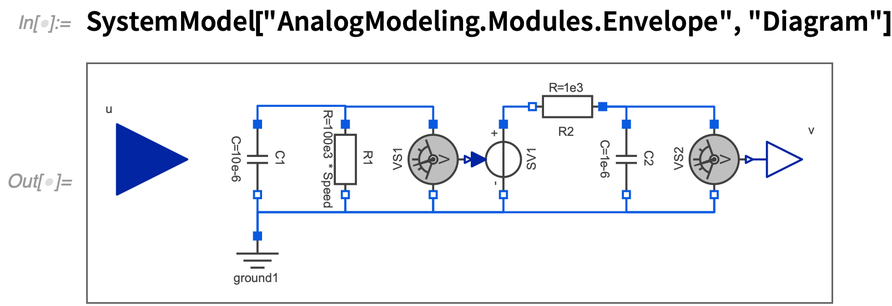✕ `SystemModel["AnalogModeling.Modules.Envelope", "Diagram"]`

In the previous diagram, the capacitor C1 is recharged to 1 V every time the input voltage becomes larger than 0.5 V. This is achieved by adding the following equation to the model:The decay of the envelope is defined by changing the value of the capacitor C1. The envelope has a second RC stage that is used to smoothen the transitions. The values of the capacitors and resistors were arbitrarily chosen to provide the desired transition.

To test the envelope, we combine it with the VCA model and trigger it with a 1 Hz pulse. The audio signal is a sine wave of 440 Hz: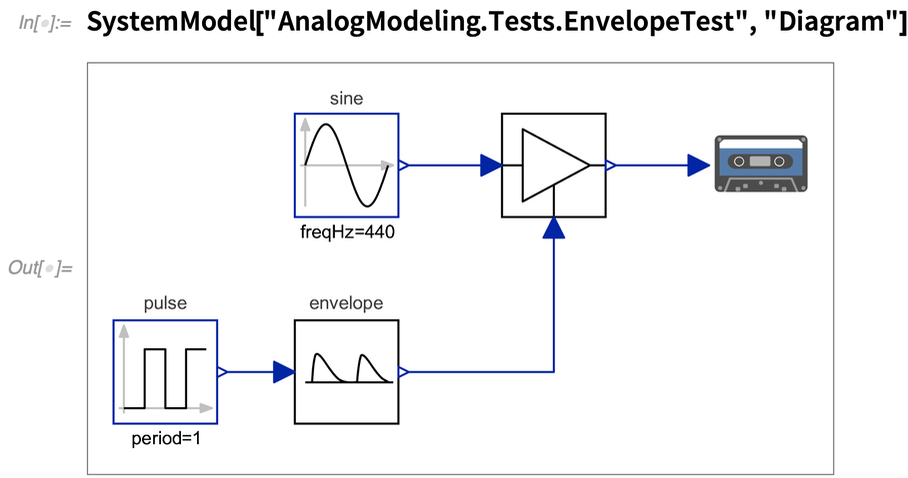✕ `SystemModel["AnalogModeling.Tests.EnvelopeTest", "Diagram"]`

In the simulation results, we can see the change of amplitude in the signal: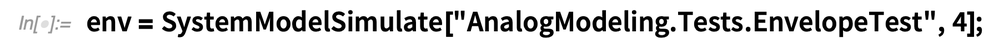✕ `env = SystemModelSimulate["AnalogModeling.Tests.EnvelopeTest", 4];`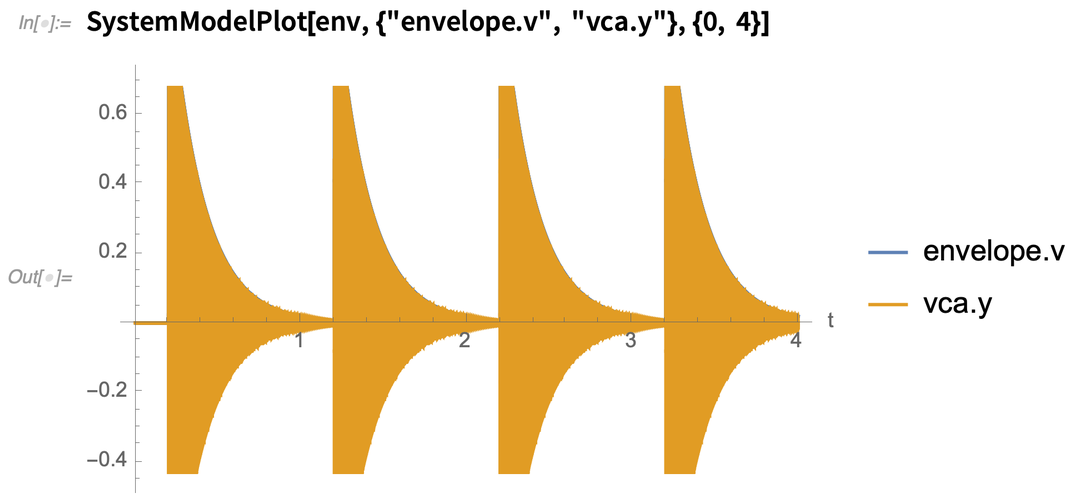✕ `SystemModelPlot[env, {"envelope.v", "vca.y"}, {0, 4}]`

Listening to the results, we can hear that this simulation sounds like gently hitting a glass of wine with your finger: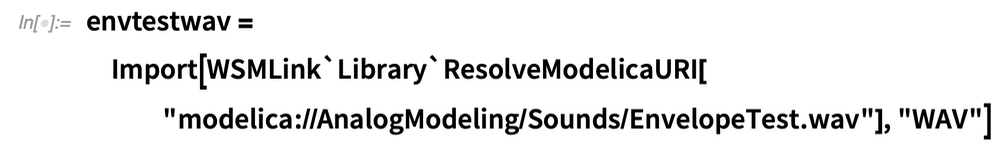✕ ```envtestwav = Import[WSMLink`Library`ResolveModelicaURI[ "modelica://AnalogModeling/Sounds/EnvelopeTest.wav"], "WAV"]```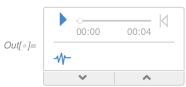Using these basic models (plus some utilities from the Modelica library), I have created the short loop of techno music that was presented in the beginning of the post. Without going into the details, this loop consist of three voices: one kick drum, one bass synthesizer and one hi-hat synthesizer. All of them are combined using a mixer (summing voltages) and recorded directly to a WAV file: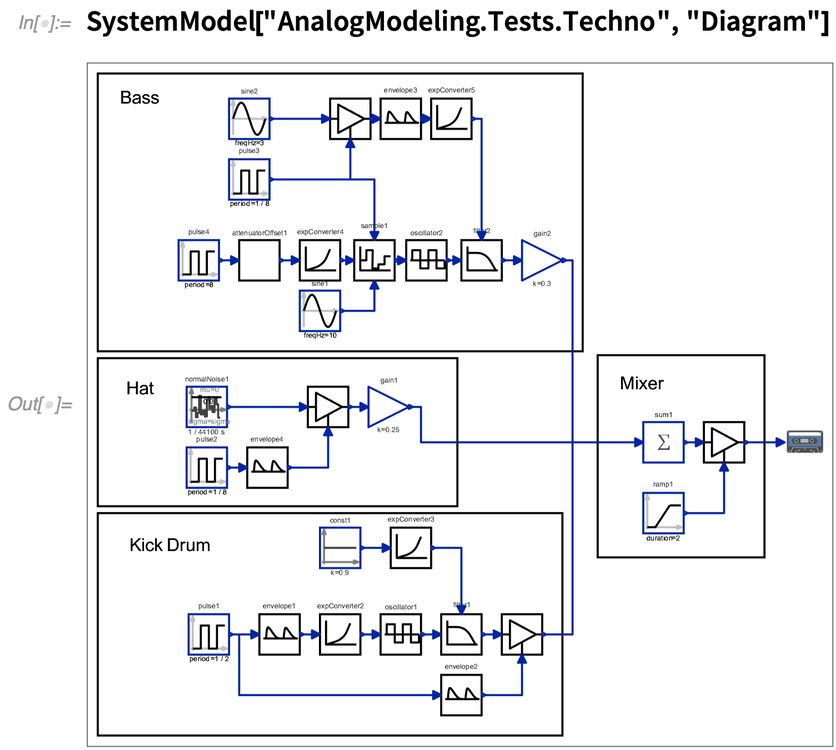✕ `SystemModel["AnalogModeling.Tests.Techno", "Diagram"]`

## Why Aren’t Musicians Using System Modeler to Perform Live?

The previous example takes about 70 seconds to render 30 seconds of audio. This is more than two times slower than real time. The main reason for this is because the solvers used by System Modeler are focused on providing high-accuracy simulations. In addition, System Modeler calculates the internal voltages and currents of every component in the circuit. This is very handy when performing analysis on our models, but as I have mentioned before, when creating music we can consider all our models as black boxes and optimize them in order to consume as little CPU as possible.

When optimizing the simulation models, there are other compromises that we can make in order to obtain an efficient simulation. One of these things is to simulate the models with less accurate methods. This will result in a simulation that is not perfect, but it will be faster and the listener will have a difficult time noticing which simulation is which. It is a matter of finding a good tradeoff between the quality of our model/simulation and the efficiency. If the simulation is oversimplified, we may lose the “analog vibes” of the sound. On the other hand, if the simulation is very accurate, the users will consume their available CPU resources quickly.

## Implementing the Filter as an Audio Plugin

Now we are going to implement the Sallen–Key filter equations we obtained and use the filter to process audio inside VCV Rack. In order to do that, we need VCV Rack and a plugin called VCV Prototype. Both are freely available from the VCV Rack website. The VCV Prototype is a special module that allows testing of our own DSP code without the need for any extra tools. It also has support for a programming language I developed called Vult. The Vult language is an easy and constrained language that I use to write all my plugins.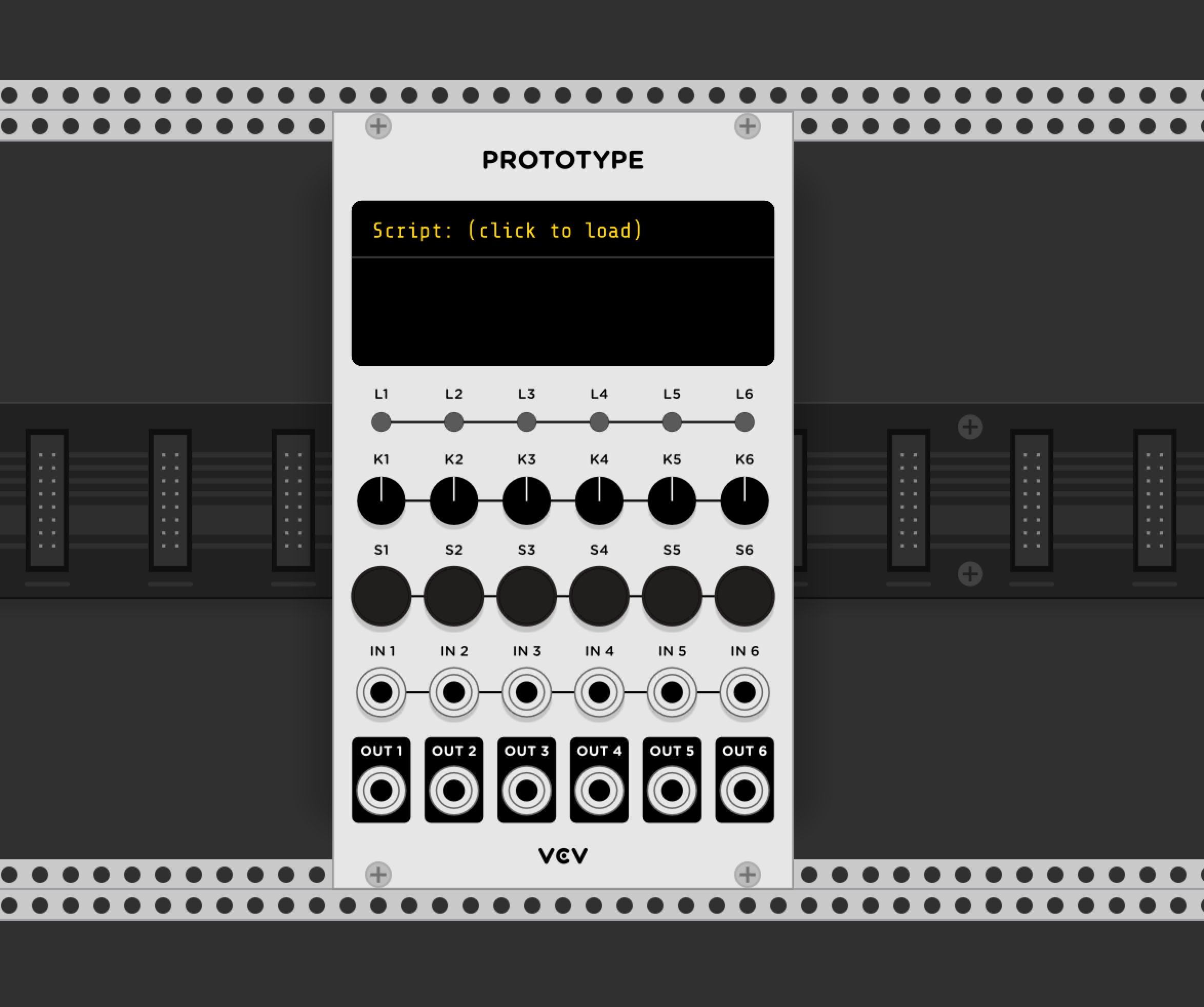VCV Rack Prototype module.

In order to implement our filter, we are going to take the differential equations we derived and then we are going to use a numerical integrator to simulate them in real time. In this example, we will use the forward Euler method. This method is simple and fast; however, it can have drawbacks such as instability or bad accuracy. But for the purposes of this example, the method is good enough.

The following figure shows the Vult language code that simulates the Sallen–Key equations we obtained. You may notice that the code has a resemblance to JavaScript or C++ code. This code declares a function filter, which receives the input voltage vin, the potentiometer position p and the simulation time step h.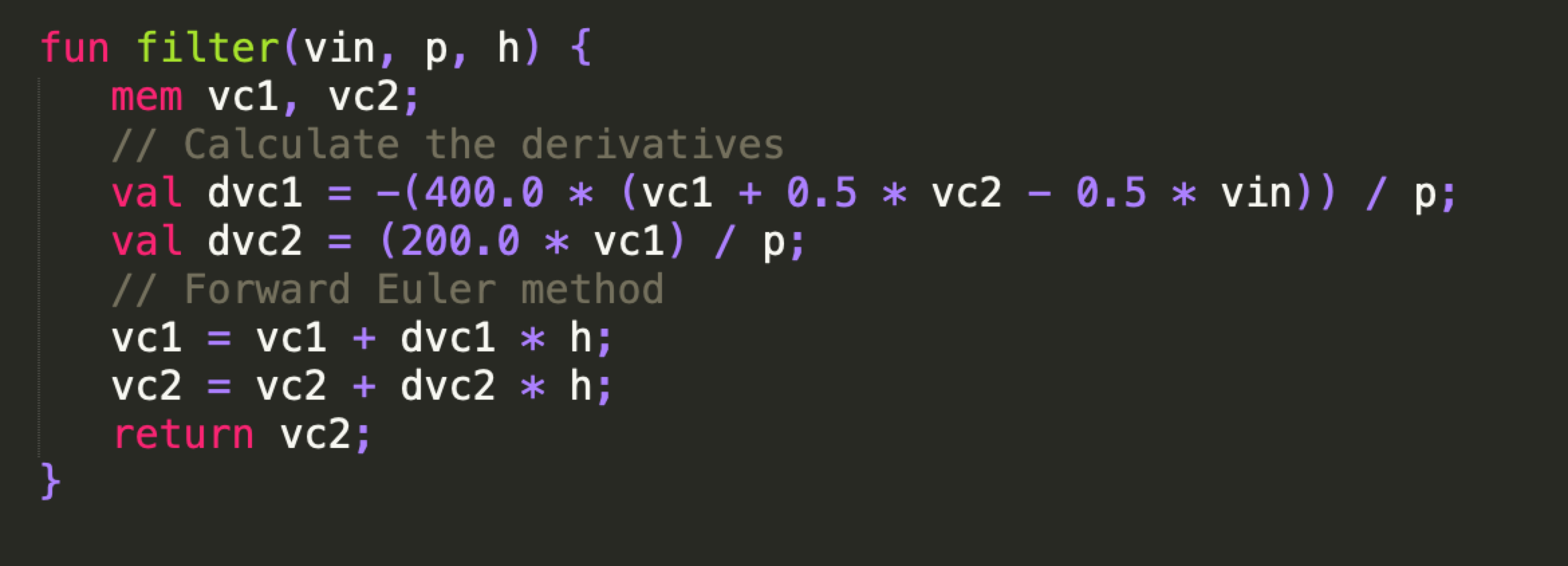The derivatives dvc1 and dvc2 are calculated, then the simulation takes one step using the Euler method. Before we can run this code, we need a small piece of code to calculate the time step h and the position of the potentiometer. There are three issues that we need to guard against before we run this code, and all of them have to do with the position of the potentiometer p. The first is that the value cannot be zero because that would produce a division by zero in the equations. Second, the value of p cannot be too small because that would make the Euler simulation method unstable. The third one is that p has a linear relation with the frequency, which is not the best when dealing with audio. In musical filters, it is preferable to control the cutoff in a musical way, which requires changing the cutoff frequency by octaves (exponentially). To deal with all these issues, we use a simple formula to calculate the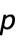parameter: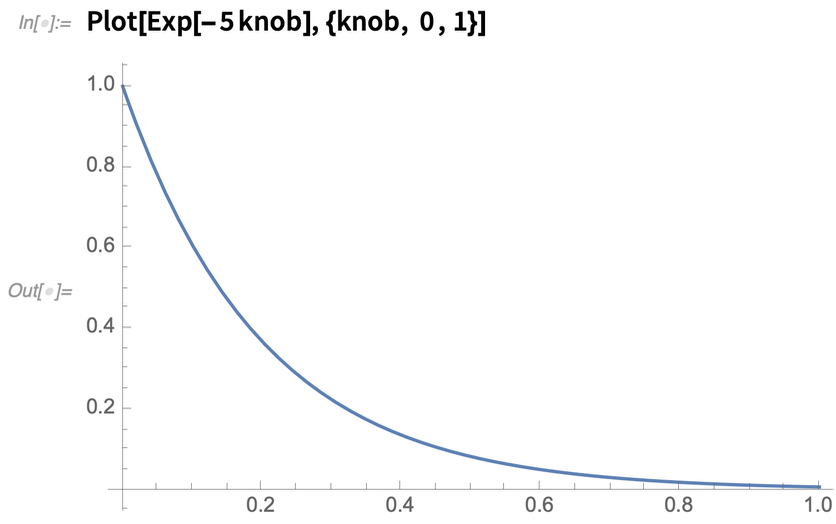✕ `Plot[Exp[-5 knob], {knob, 0 , 1}]`

This formula takes the knob variable, which represents the linear position of the potentiometer (ranging from 0 to 1) and gives us back p, which varies exponentially and never reaches zero.

If we translate this to Vult language and we run the final code in the VCV Prototype, we can finally use our filter inside VCV Rack to make music: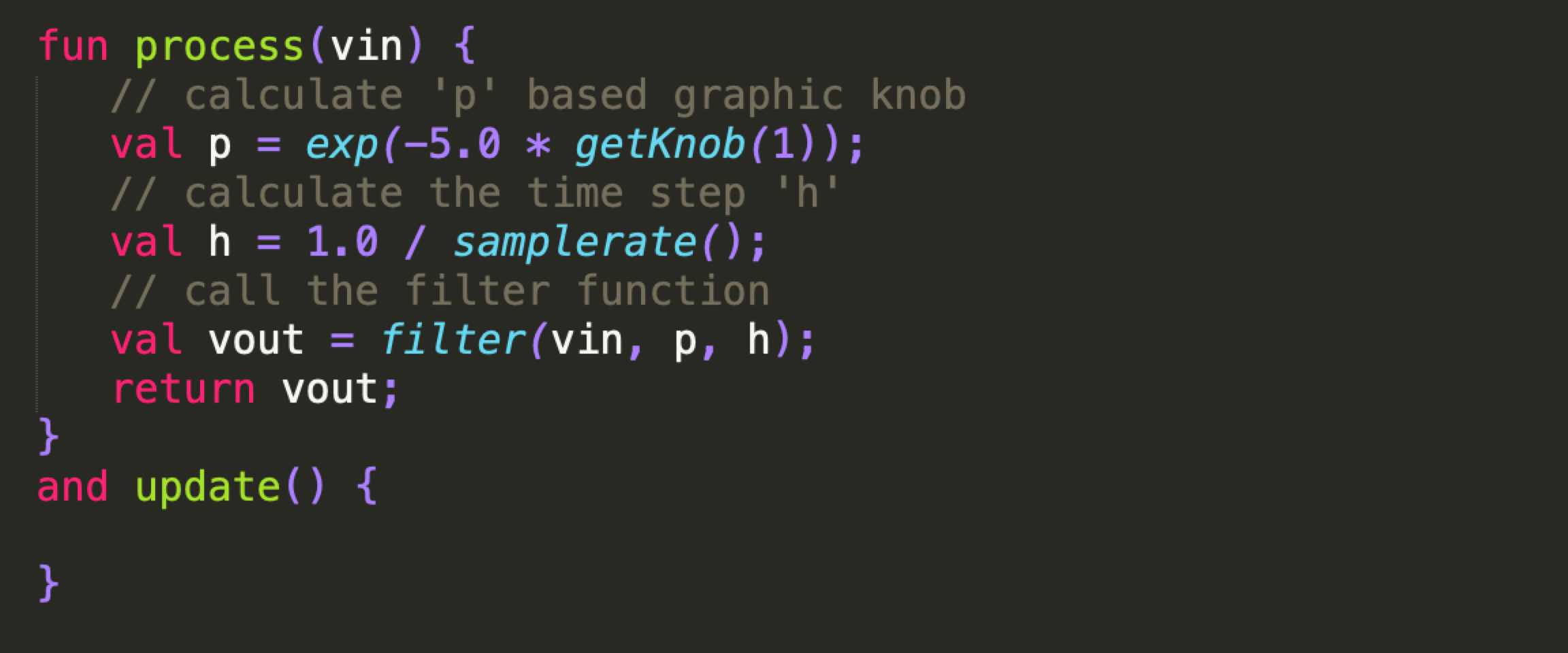In this video, you can hear the filter we implemented running in real time inside VCV Rack.

## Try It Using Wolfram Technology

Using System Modeler and Mathematica, I have modeled a large collection of analog circuits for which I have implemented more than 35 virtual modules that can be used in platforms like VCV Rack and Cherry Audio Voltage Modular.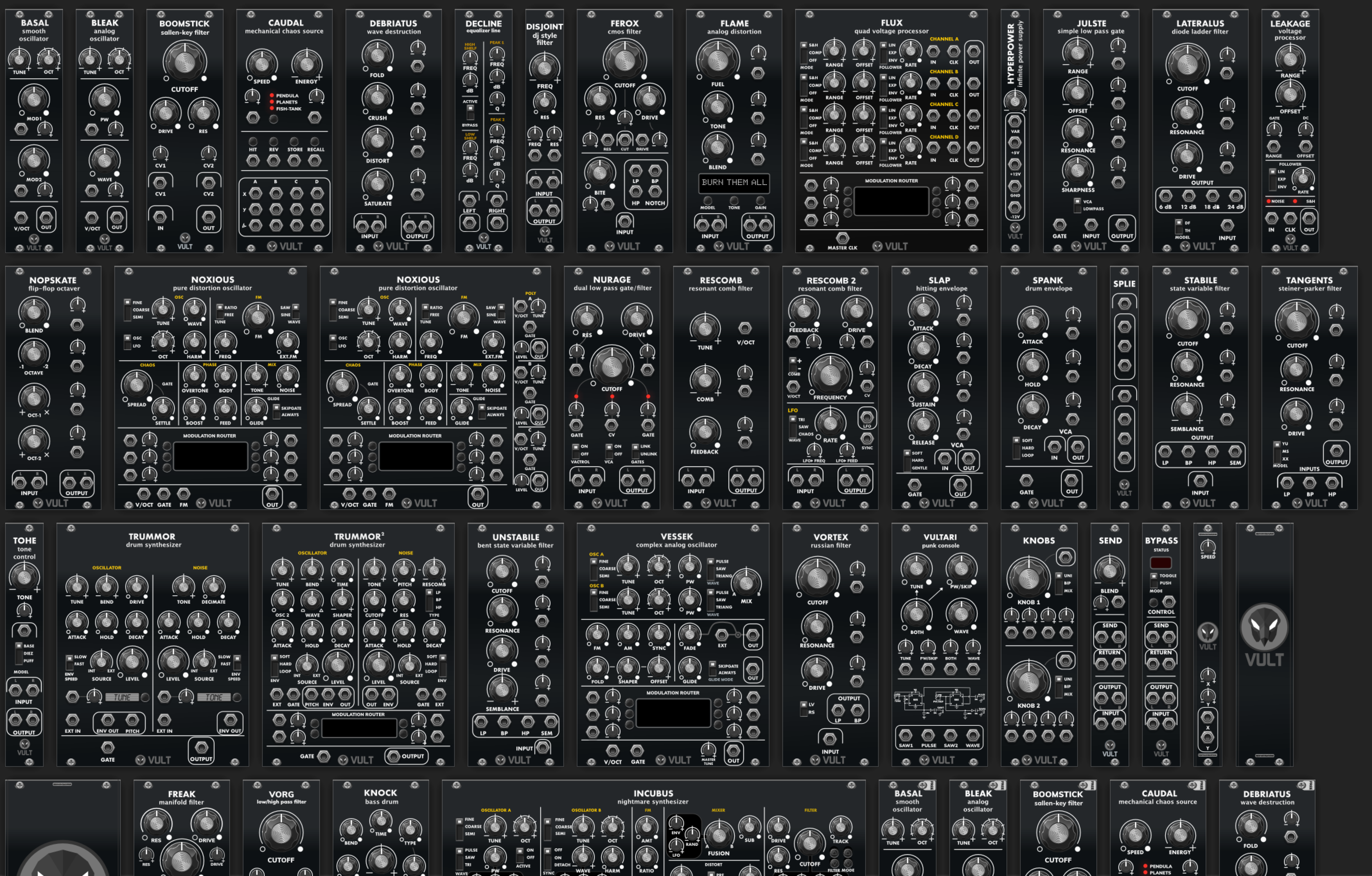Virtual models created using System Modeler.

Among all those circuits, I have modeled more than 13 analog filters used on vintage synthesizers. All those models can be run in a custom hardware module that I have designed. Now it’s possible to have the richness of sound of an analog filter, but conveniently packed into a fully digital module.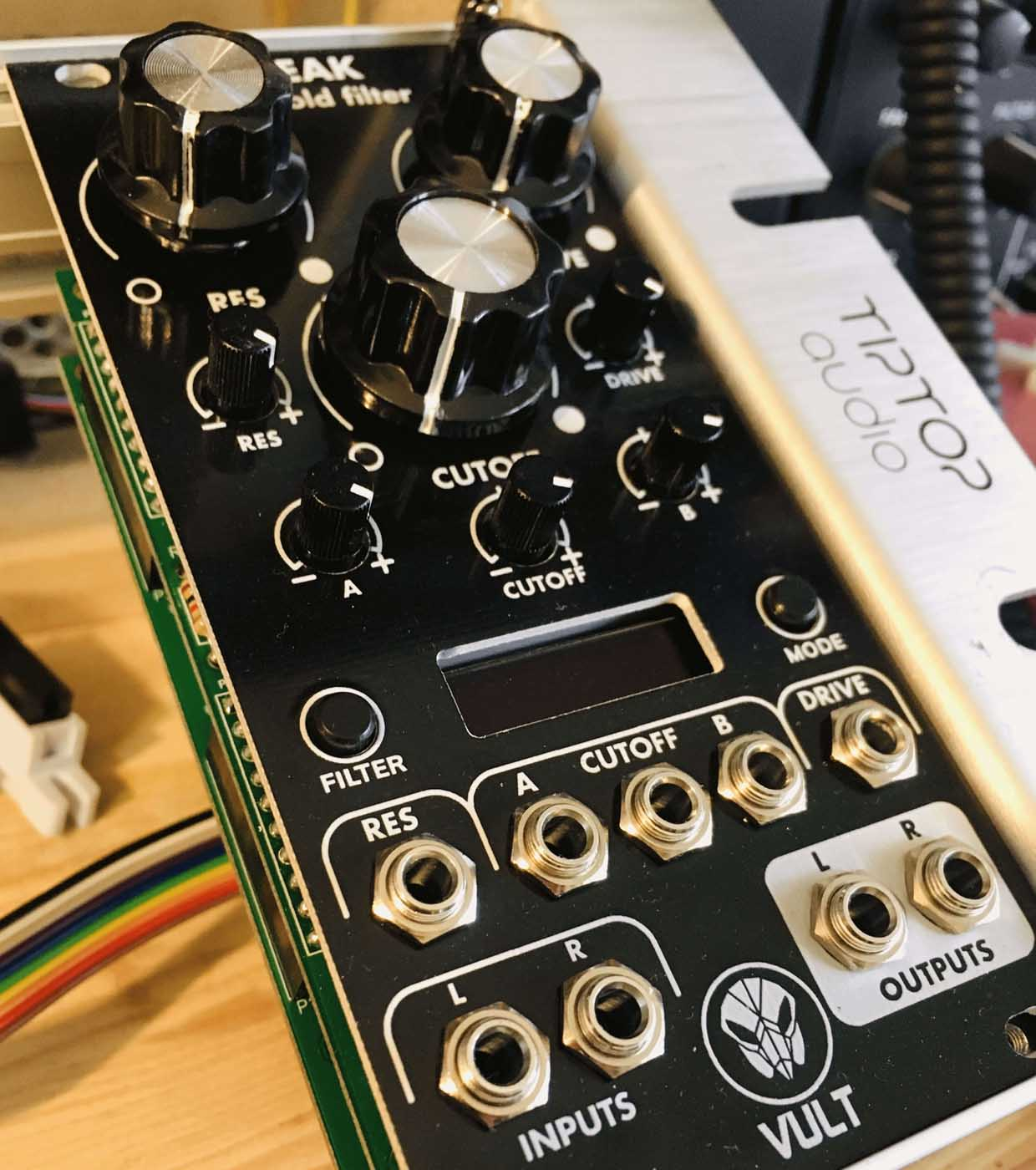An example of a custom hardware module.

I have made a complete version of this library available. You can download it to test for yourself. You can always download an unrestricted 30-day trial of System Modeler 12.1 and modify the included models to your own liking, or create your very own models for exploration, learning or advanced analysis.

For more details on what’s new in Wolfram System Modeler, visit the What’s New page or explore existing libraries at System Modeler’s library store.